# NCERT Exemplar Class 8 Maths Solutions for Chapter 7 - Algebraic Expressions And Identities Factorisation

NCERT Exemplar Class 8 Maths Chapter 7 Algebraic Expressions and Identities & Factorisation, presented here for students to prepare for exams. These exemplars solutions are designed by experts in accordance with CBSE syllabus(2018-2019), which covers all the topics of standard 8, Maths chapter 7.

This chapter is divided into two parts the first part of the chapter is about algebraic expressions and the second part is about factorization. In the first part, the students will learn about different types of algebraic expressions and their identities. In the second part, the students will learn how to factorize given equations. To learn the concepts in an easy way students are advised to solve the problems provided in the class 8 NCERT exemplar for chapter 7 algebraic expressions and identities & factorisation.

## Class 8 NCERT Exemplar For Algebraic Expressions and Identities & Factorisation

To understand the concepts present in each chapter of Maths as well as Science subject, solve NCERT exemplars for class 8, provided here. Let us discuss the topics based on which exemplars are given for chapter 7.

• Know about expressions, terms, factors, coefficient, Monomials, Binomials and Polynomials, Like and Unlike Term
• Operations performed on Algebraic Expression
• Identities of algebra and its applications
• Learn to find factors of natural numbers, algebraic expressions, by the method of common factors, by regrouping terms, using identities.
• Division of Algebraic Expressions
• Dividing monomial by monomial
• Dividing polynomial by monomial, etc.

Apart from these exemplars, students are also made available with exemplar books, NCERT solutions for 8th standard Maths and question papers to help students practice well for final exams. Students are advised to solve sample papers and previous year question papers which gives an idea of types of questions asked in the board exam from Algebraic expressions and identities and factorisation.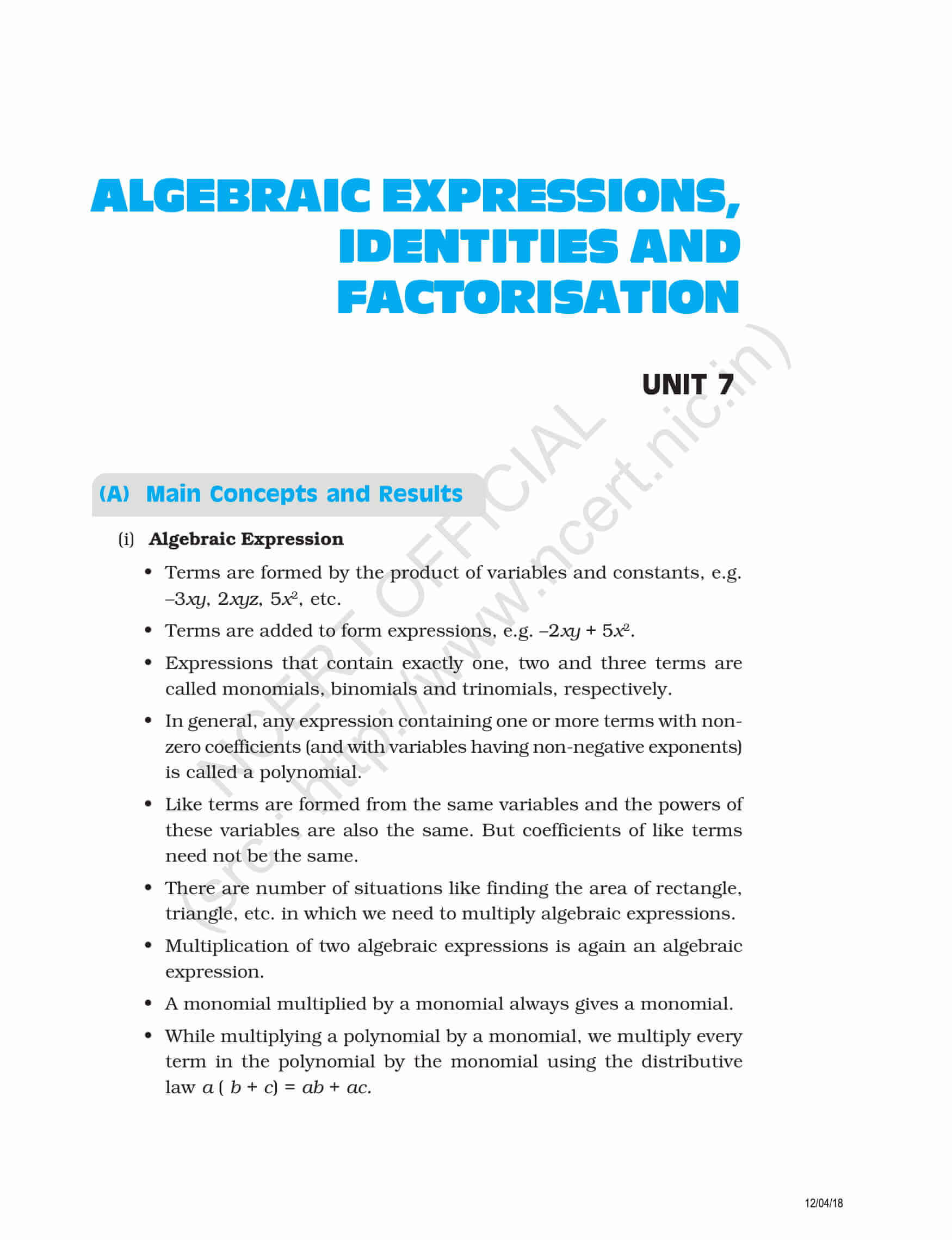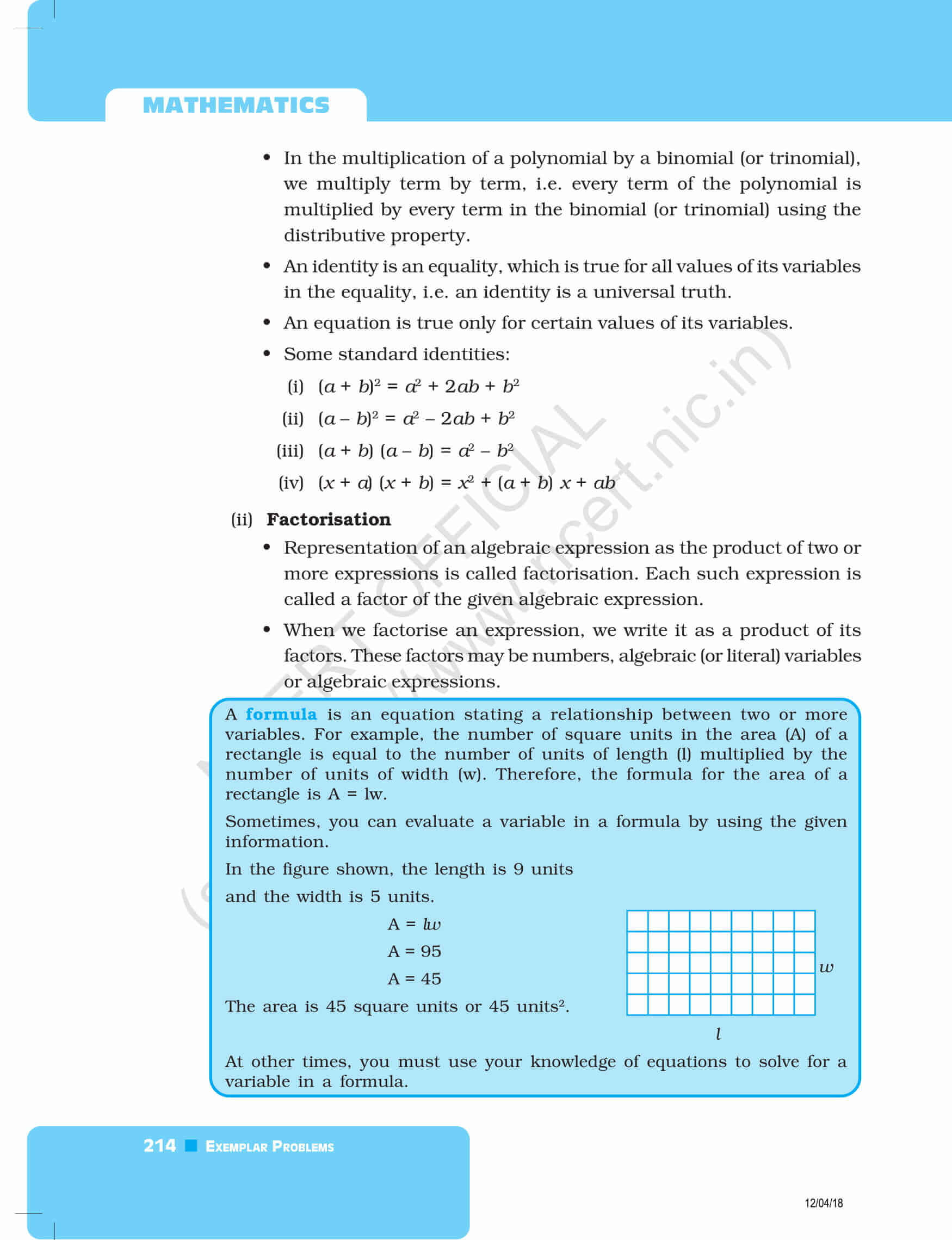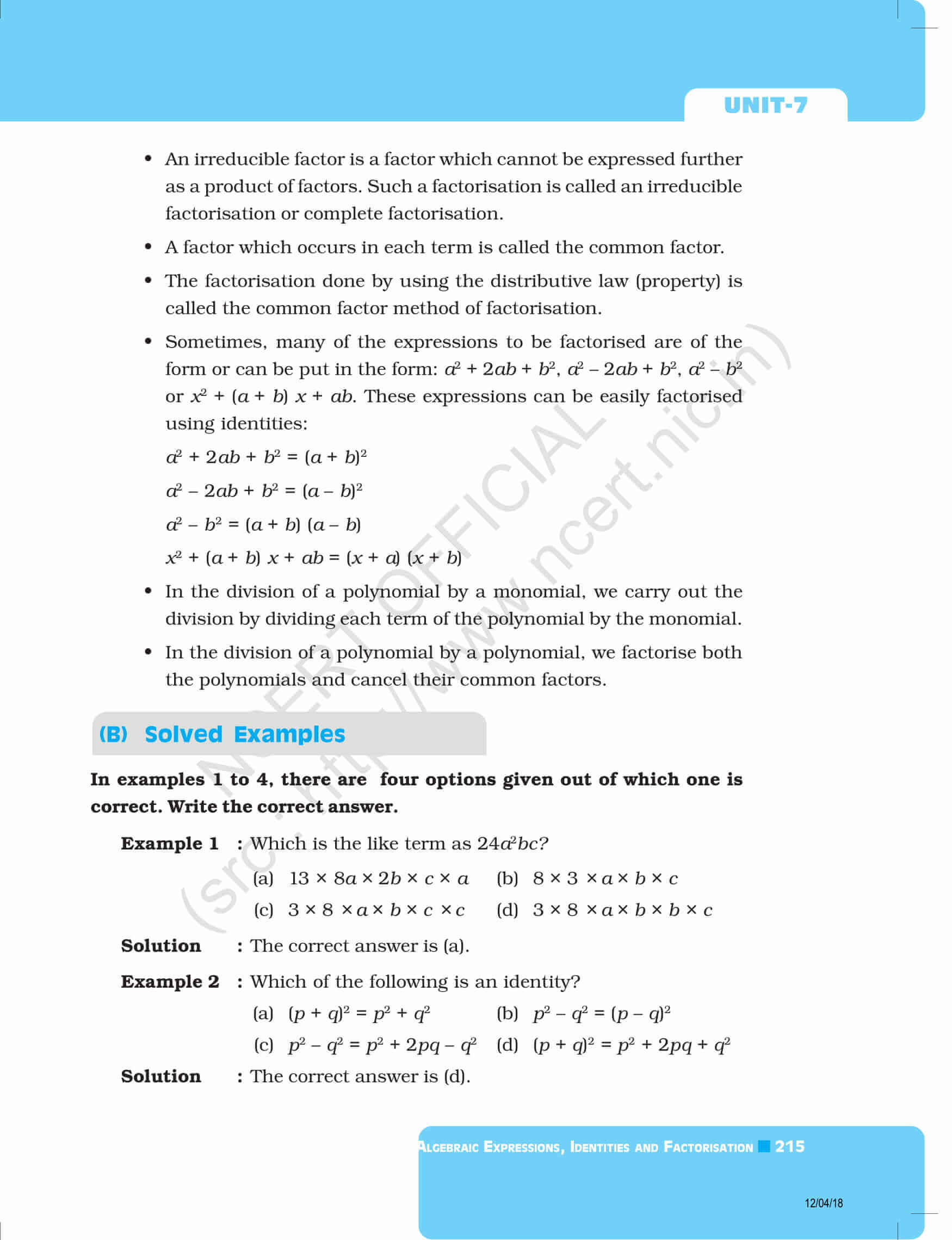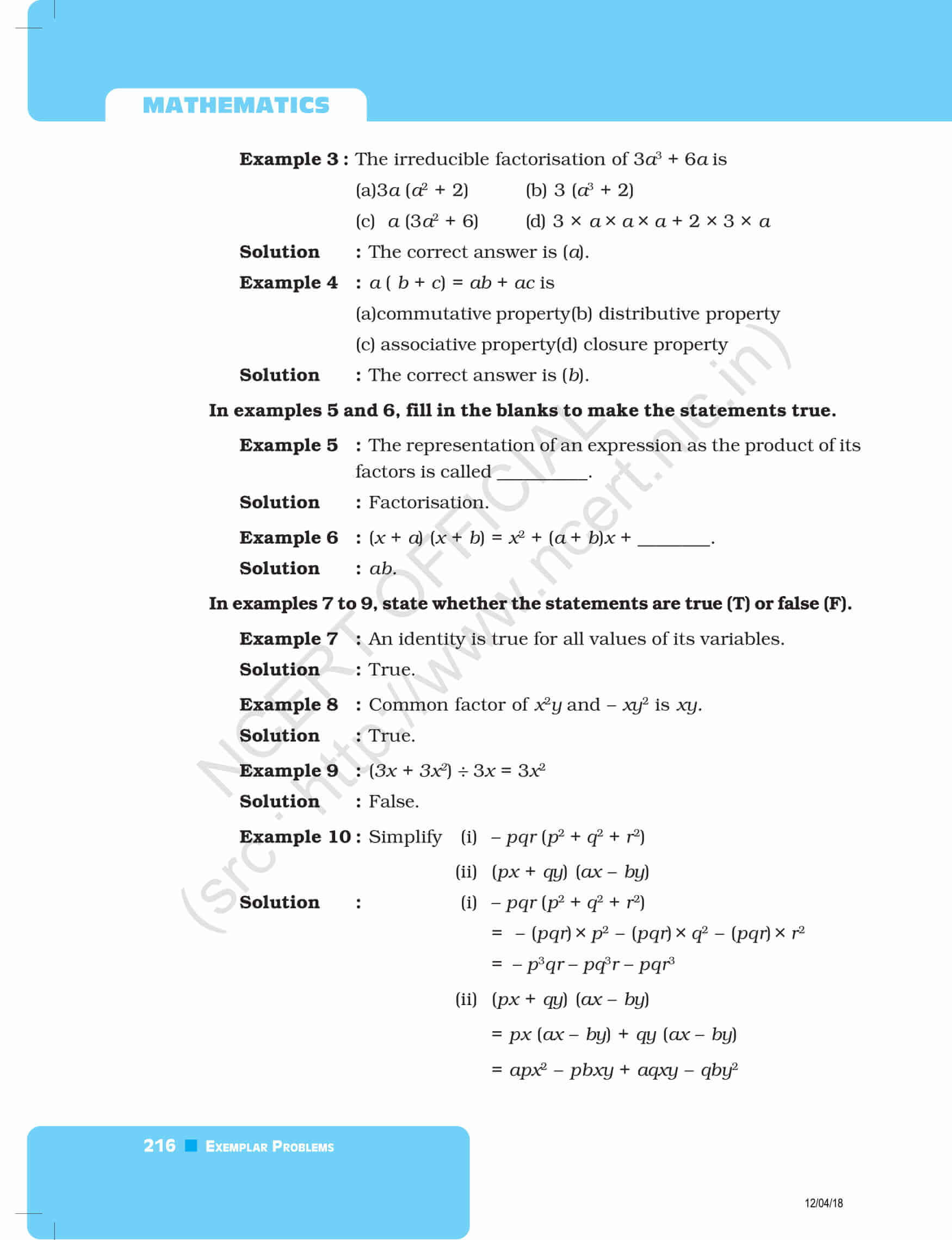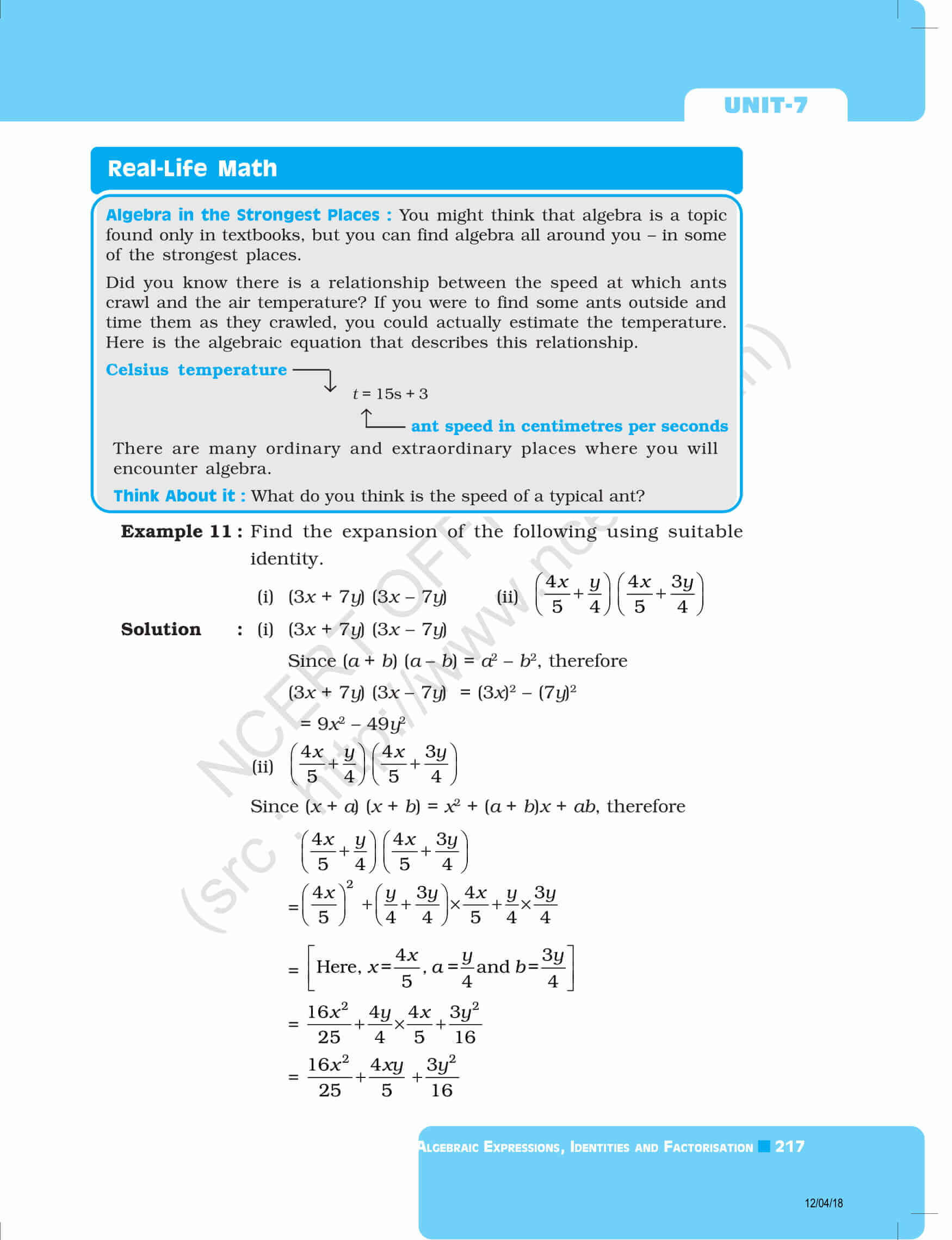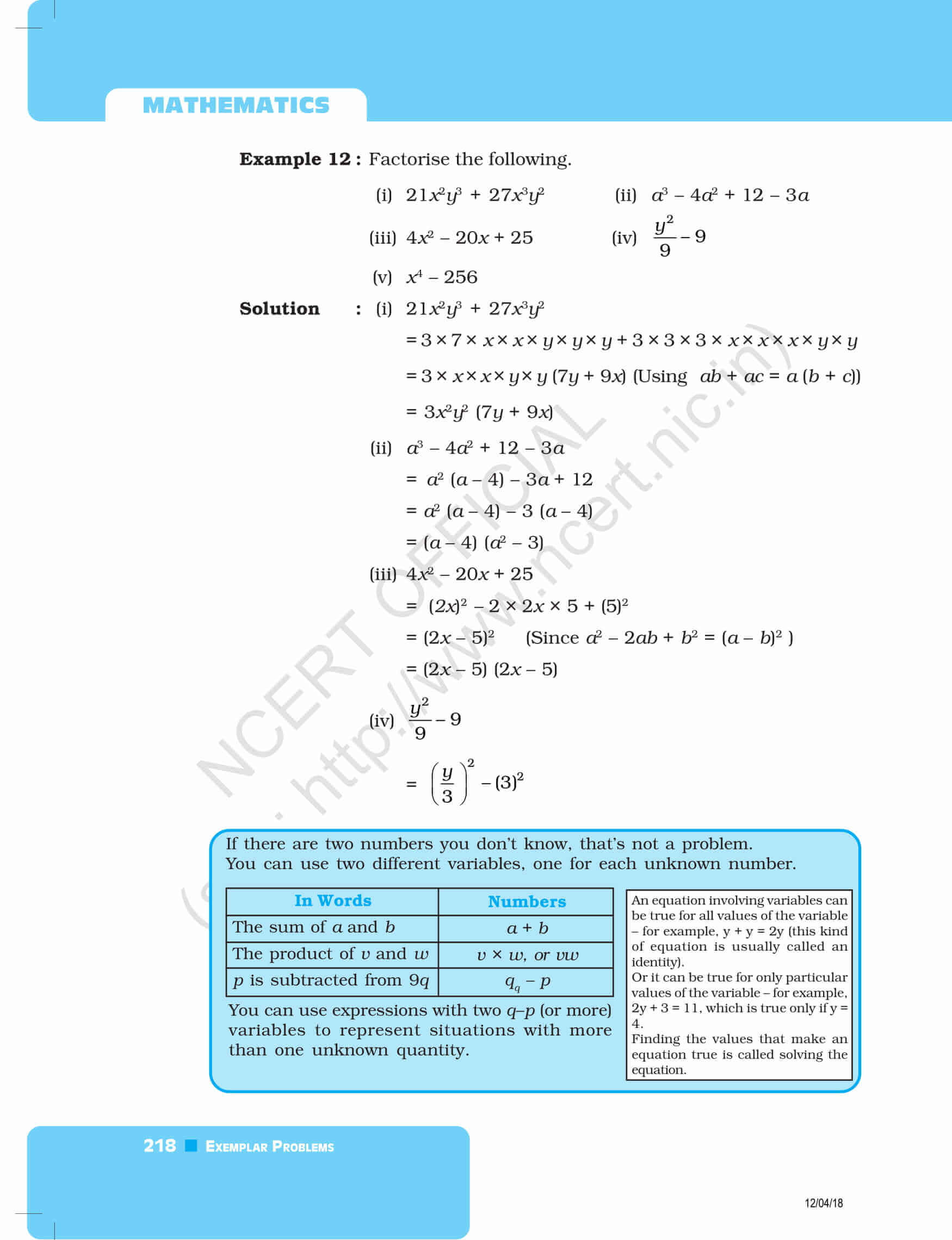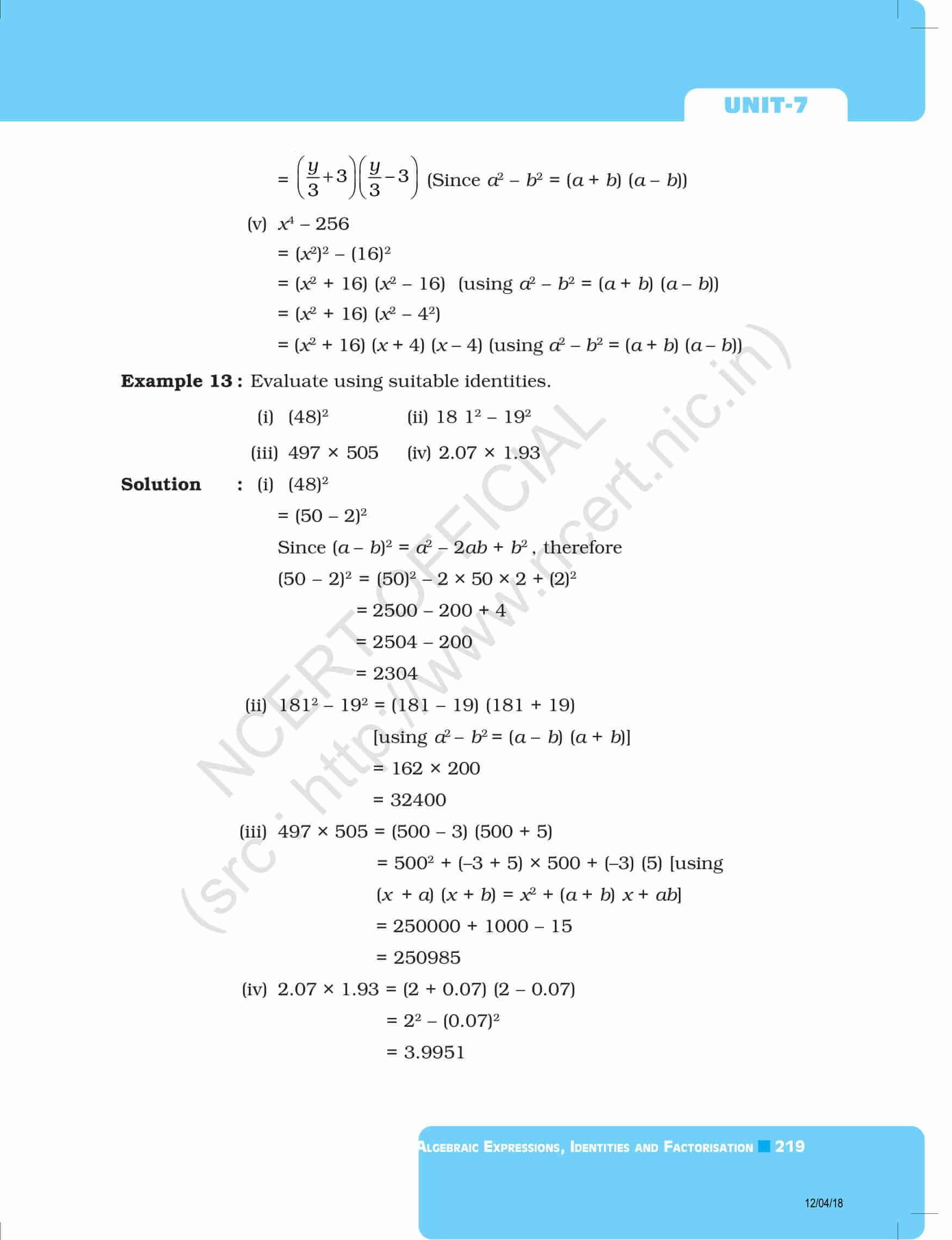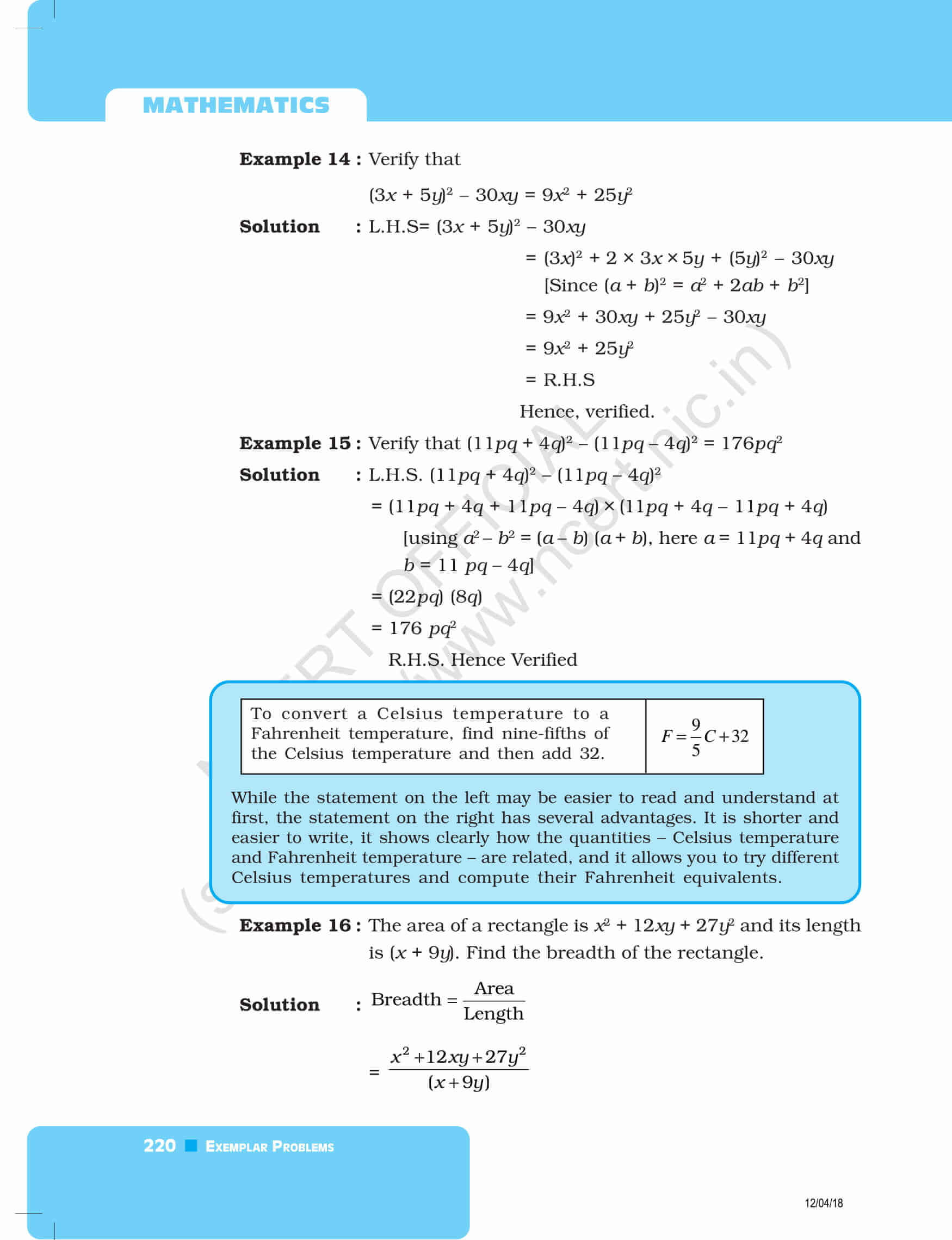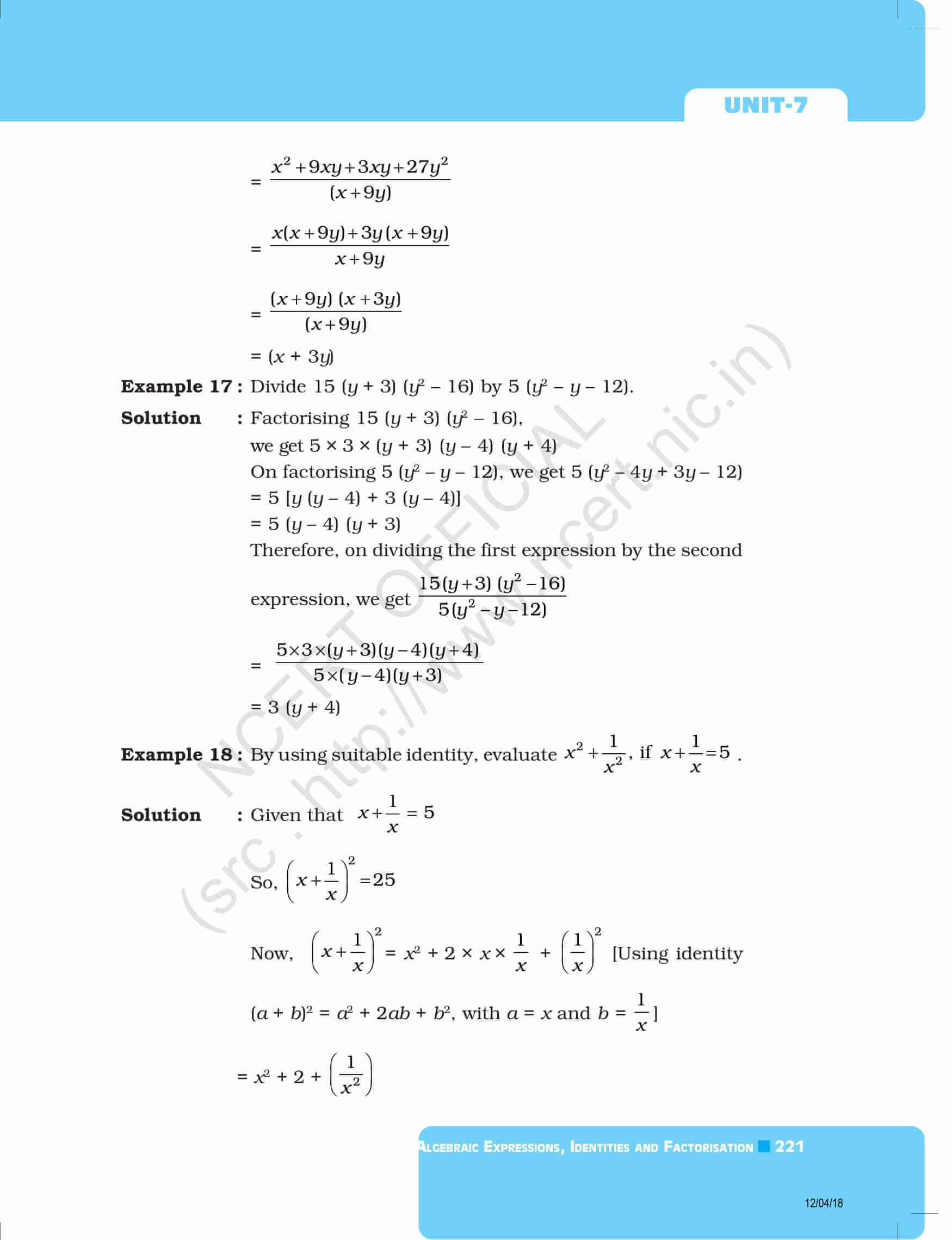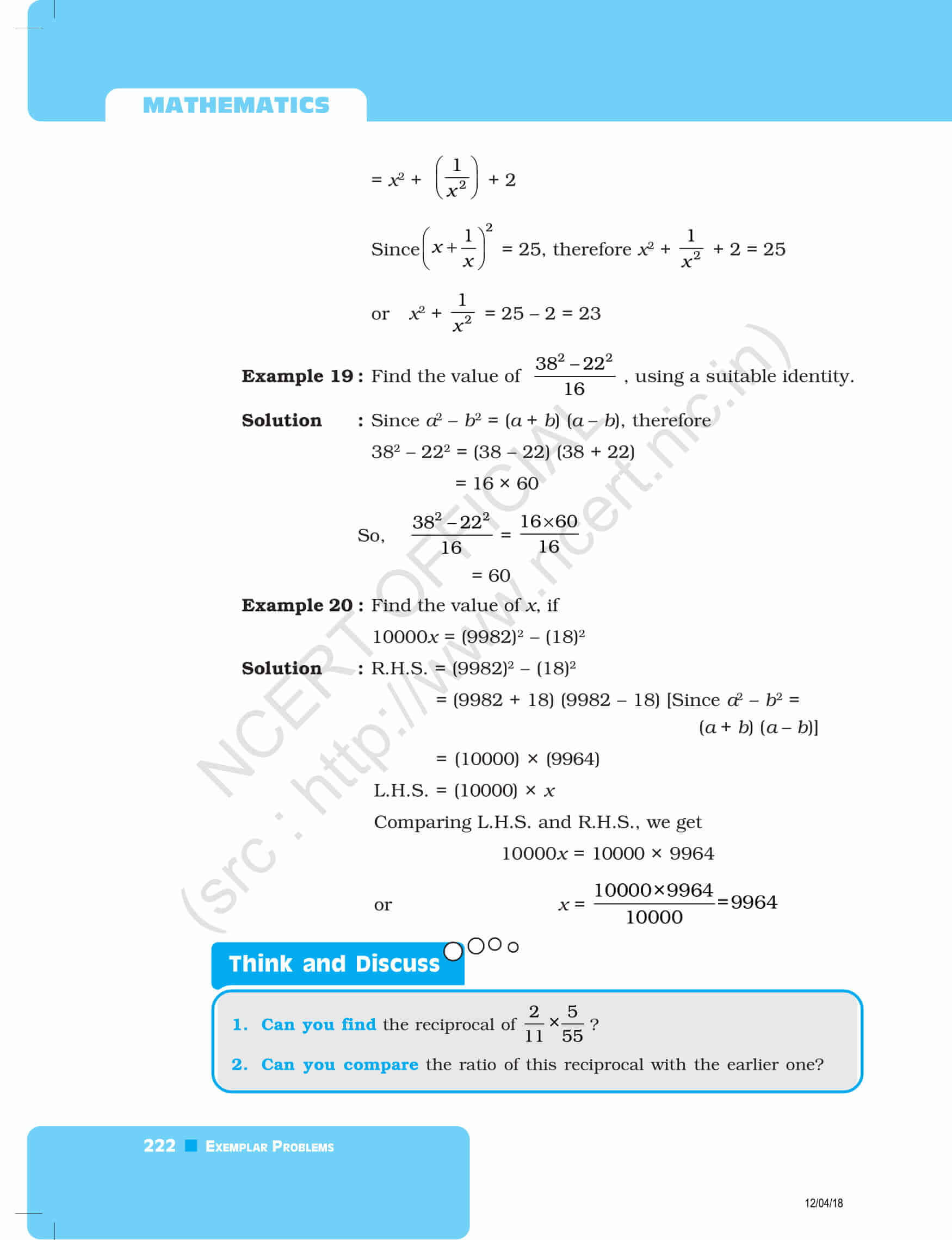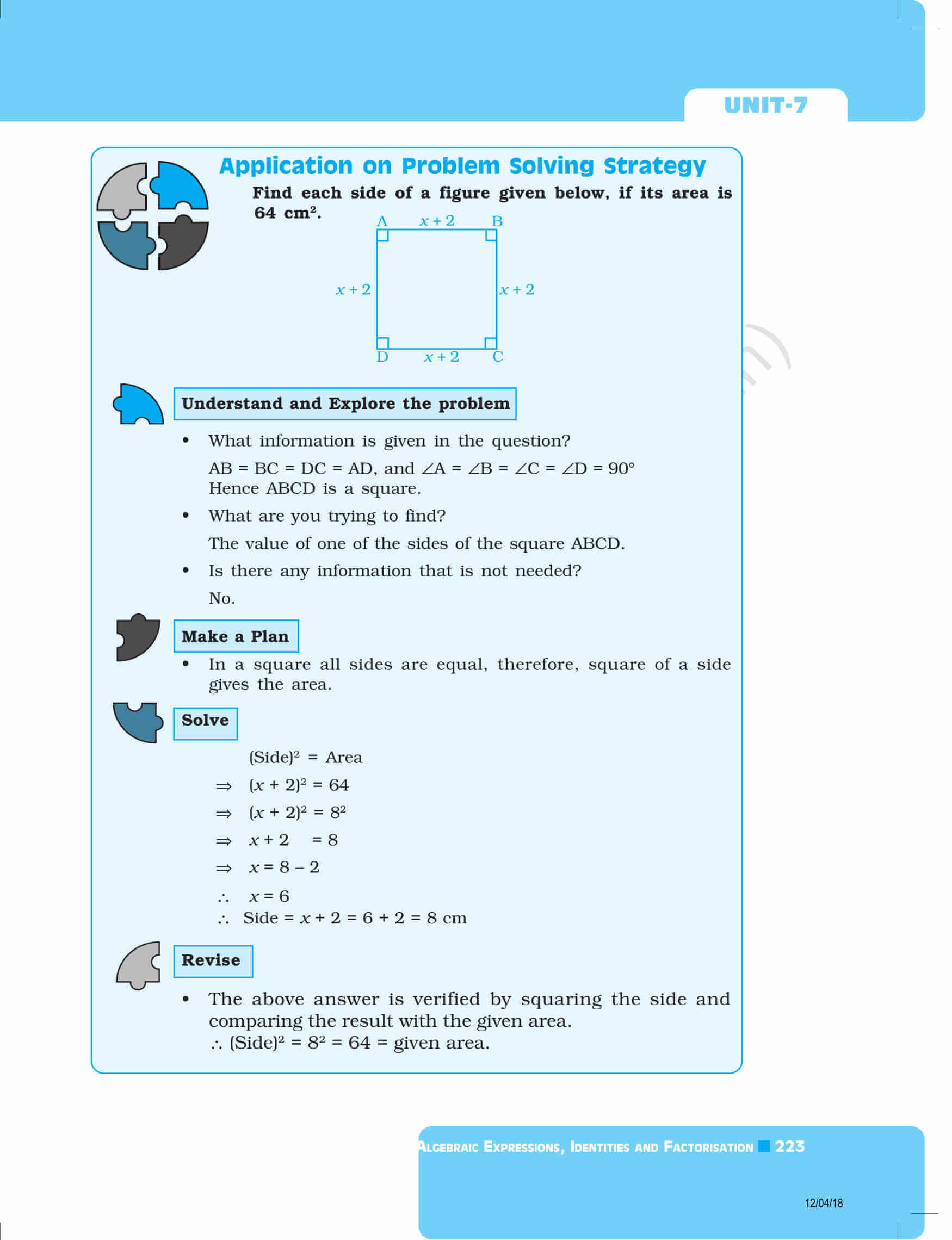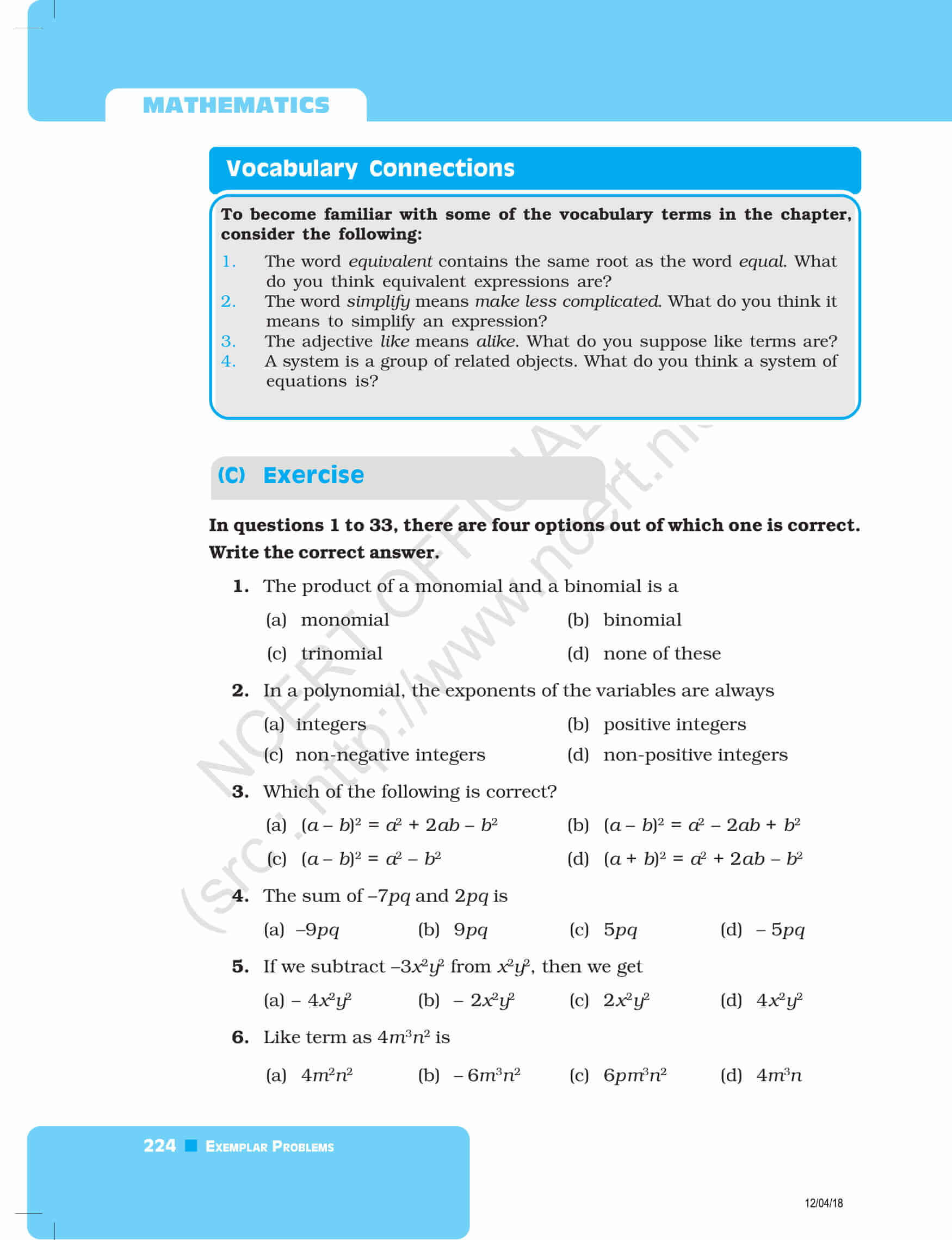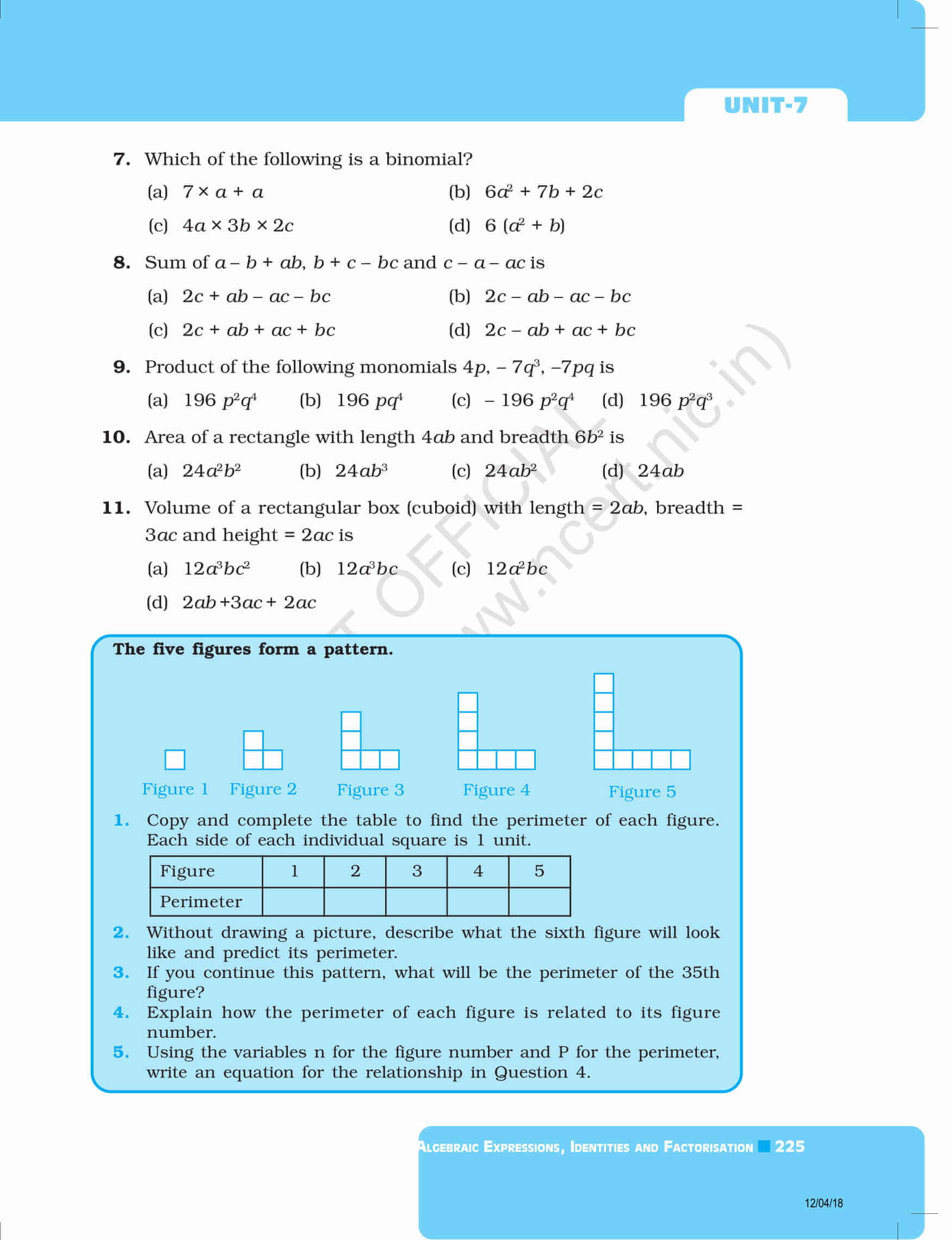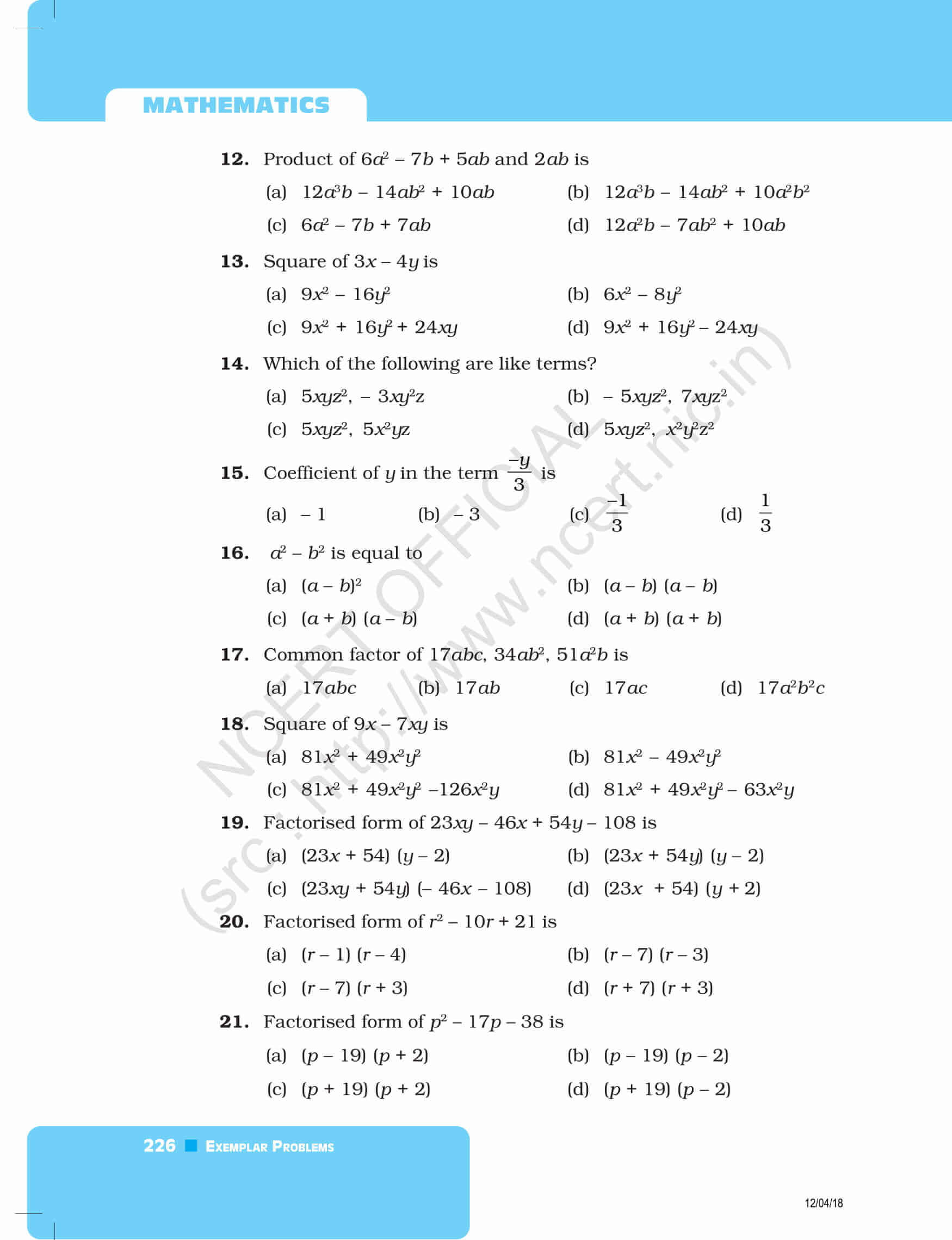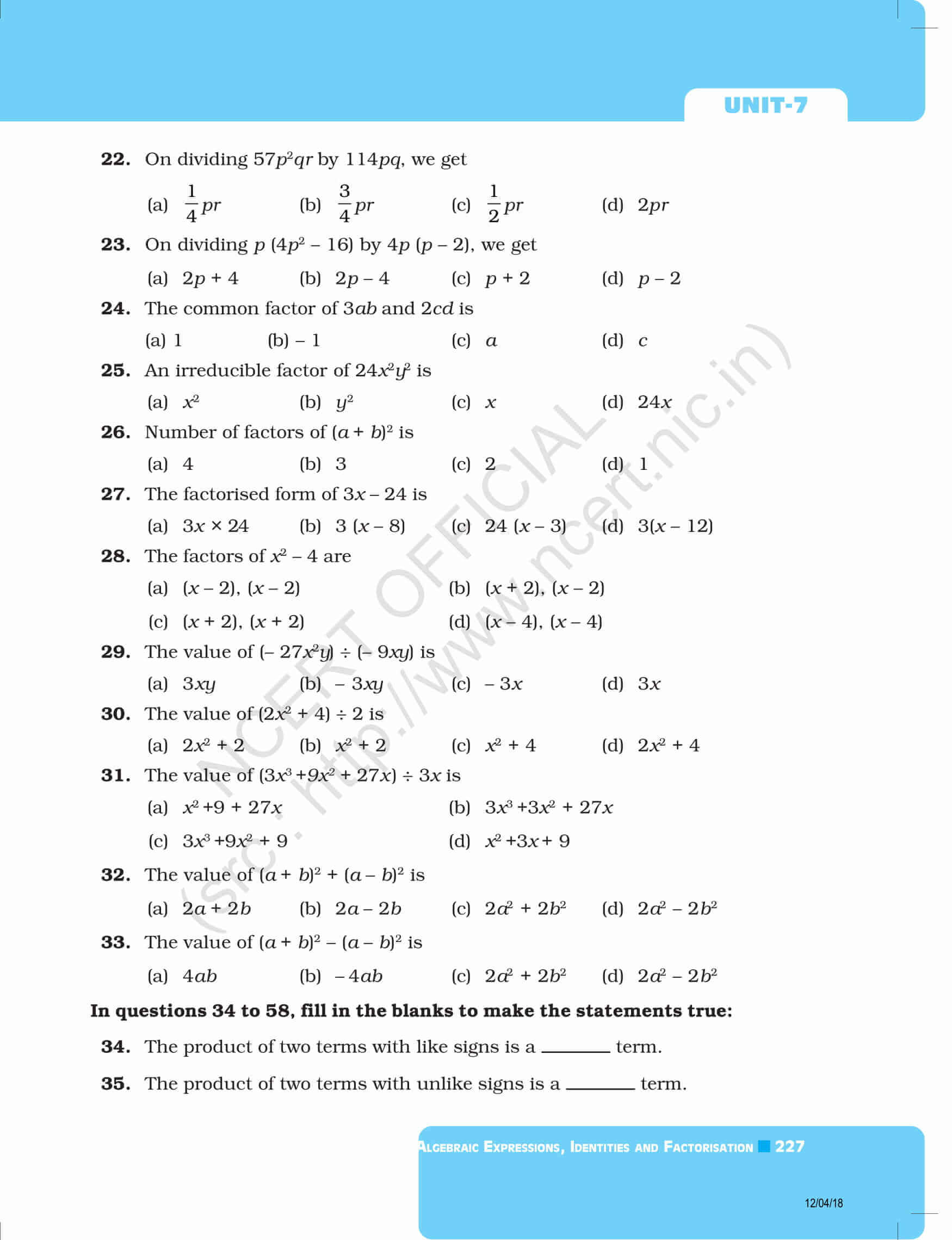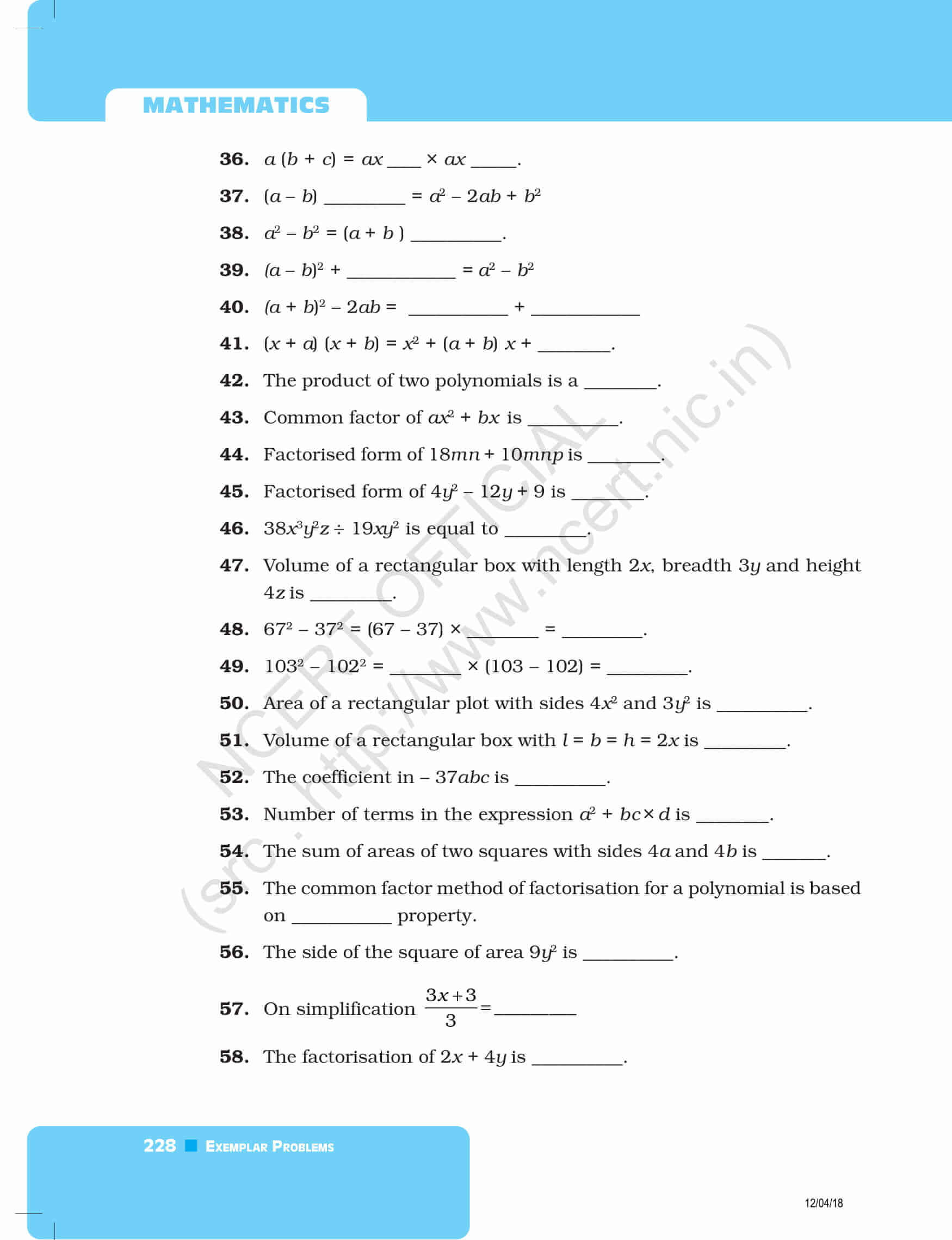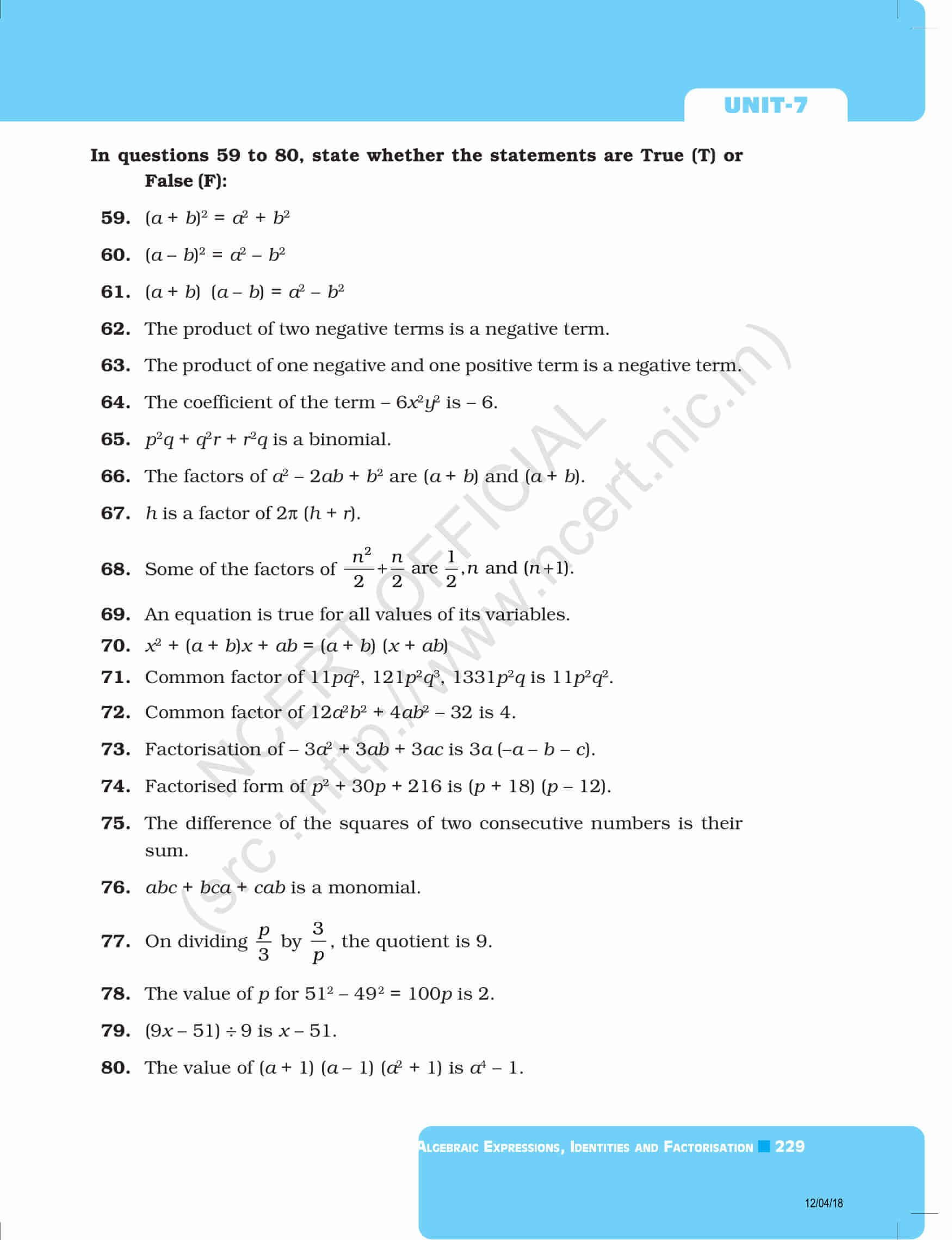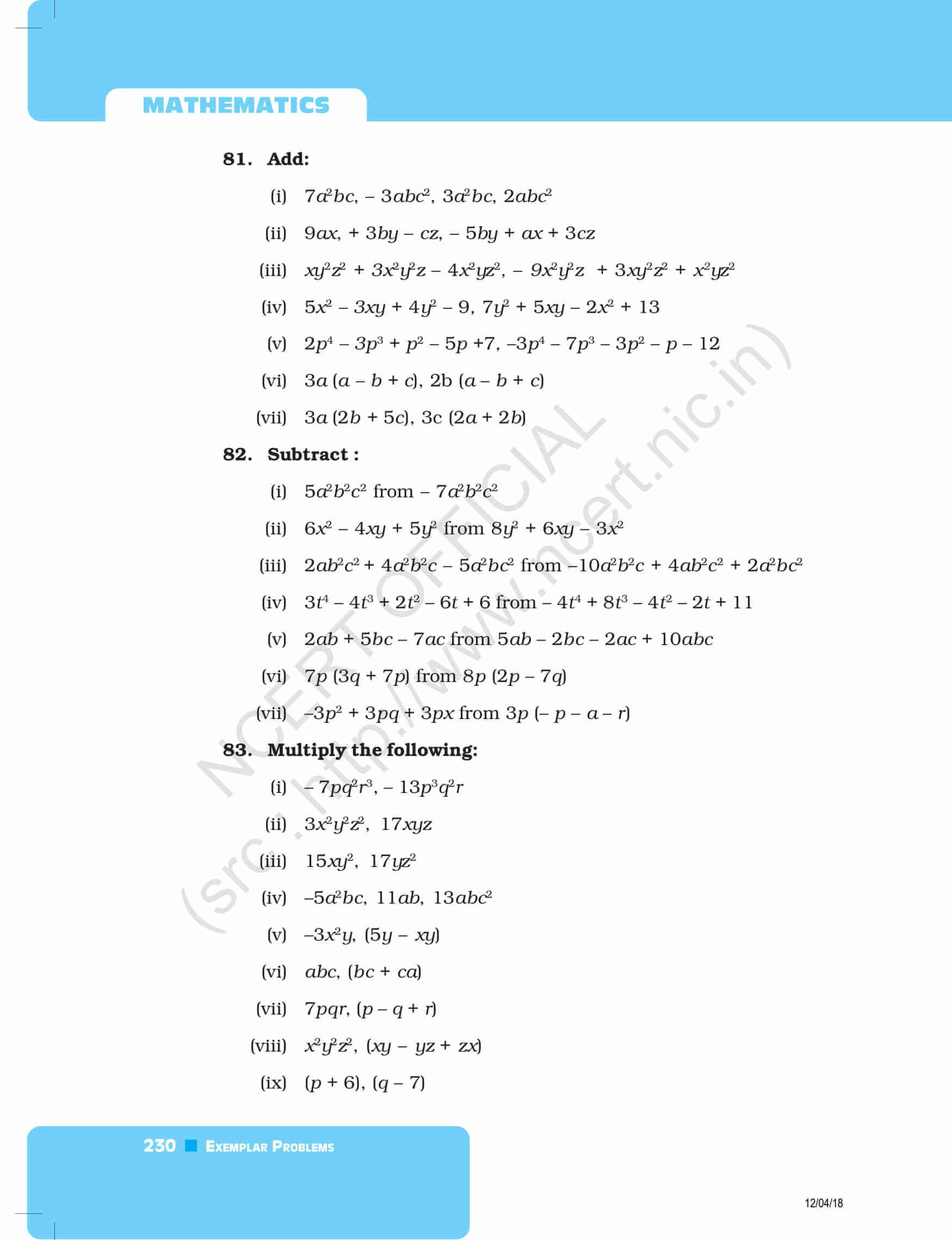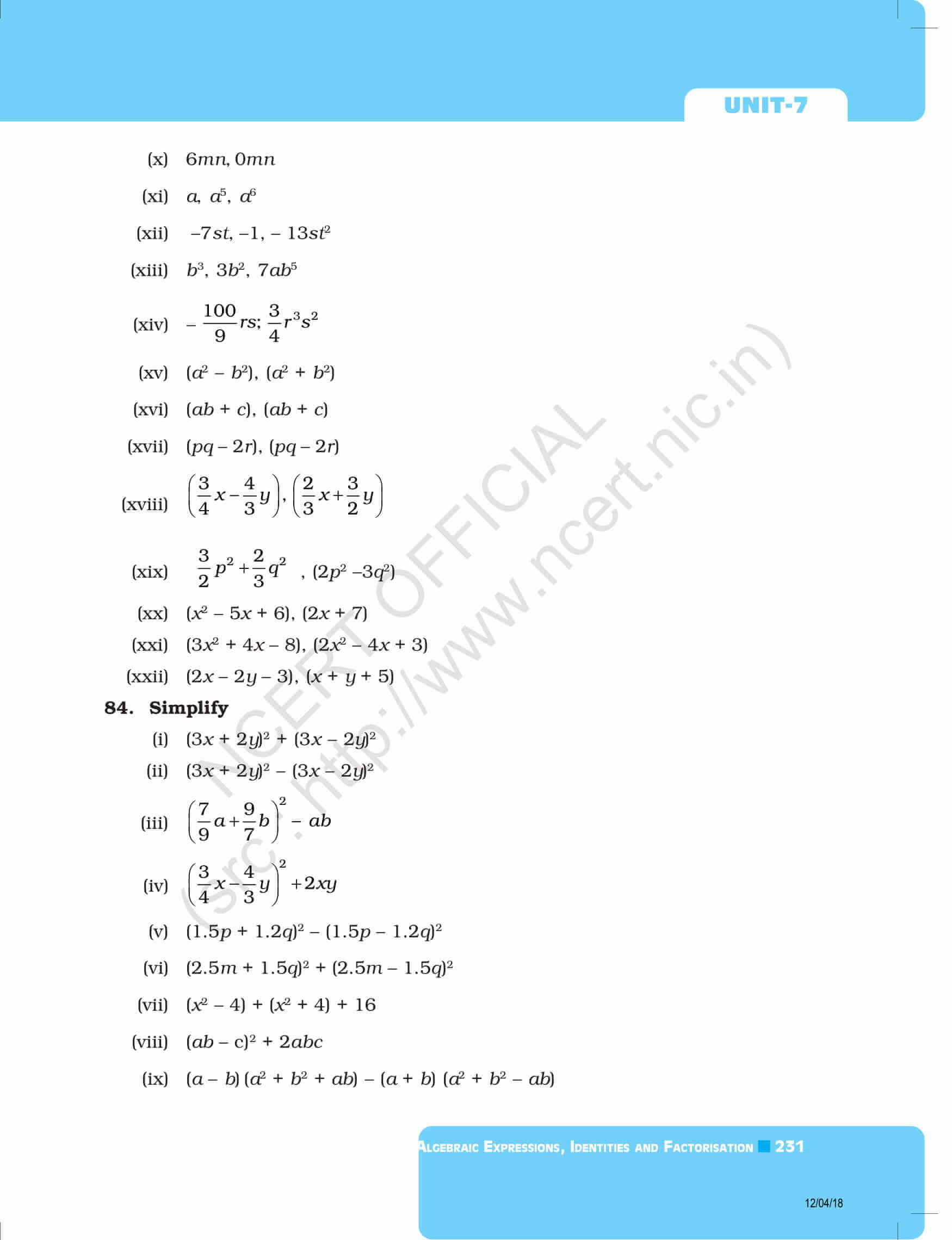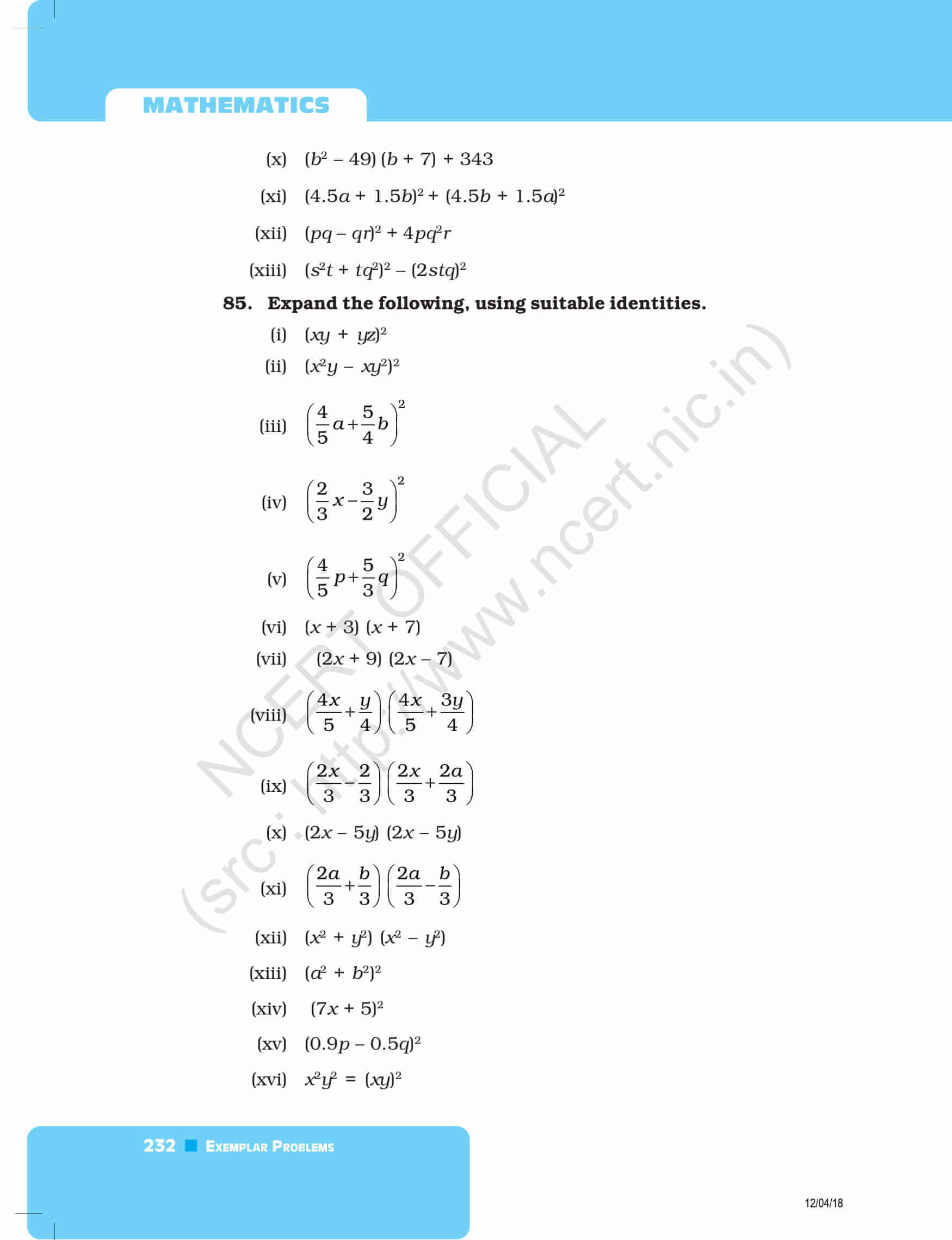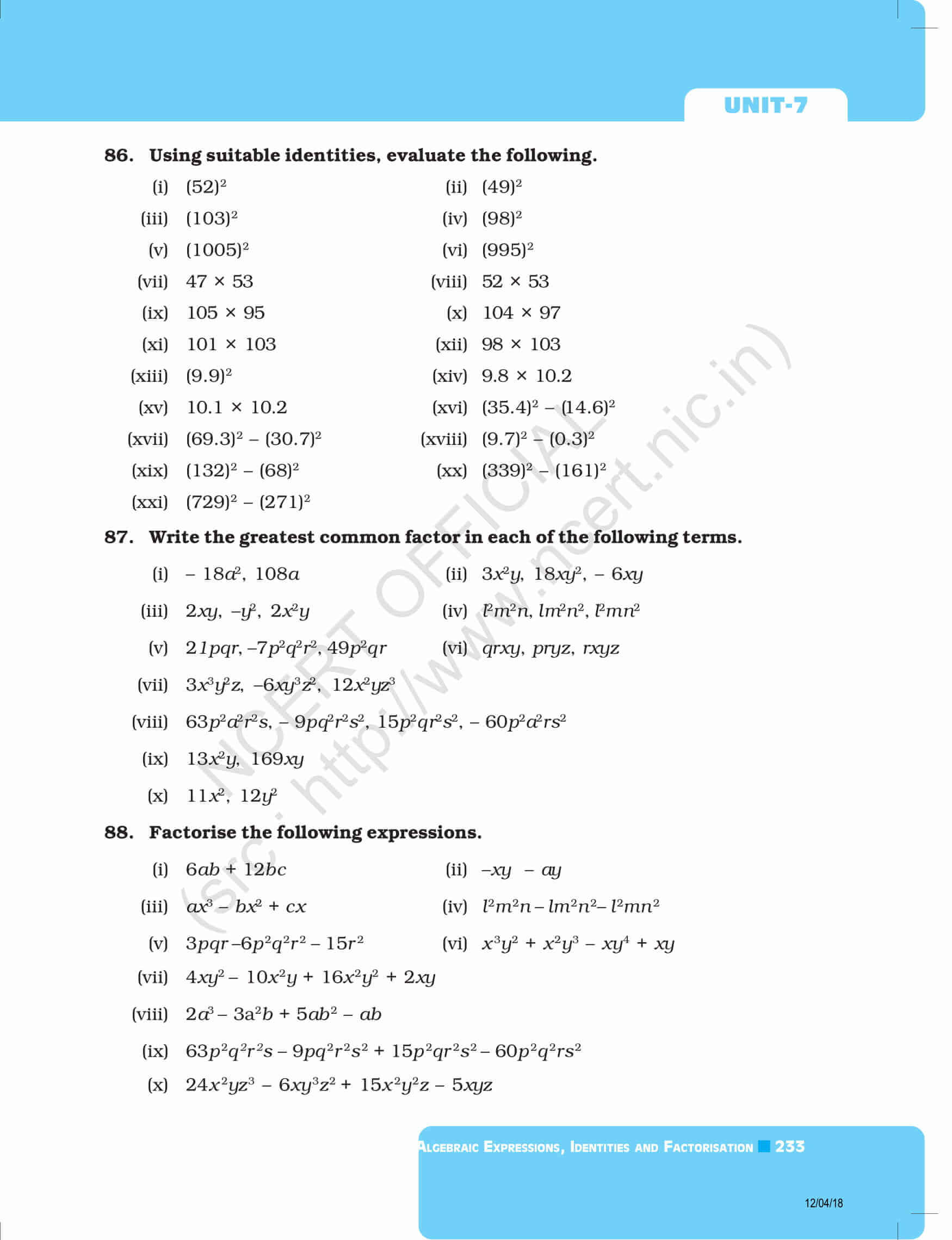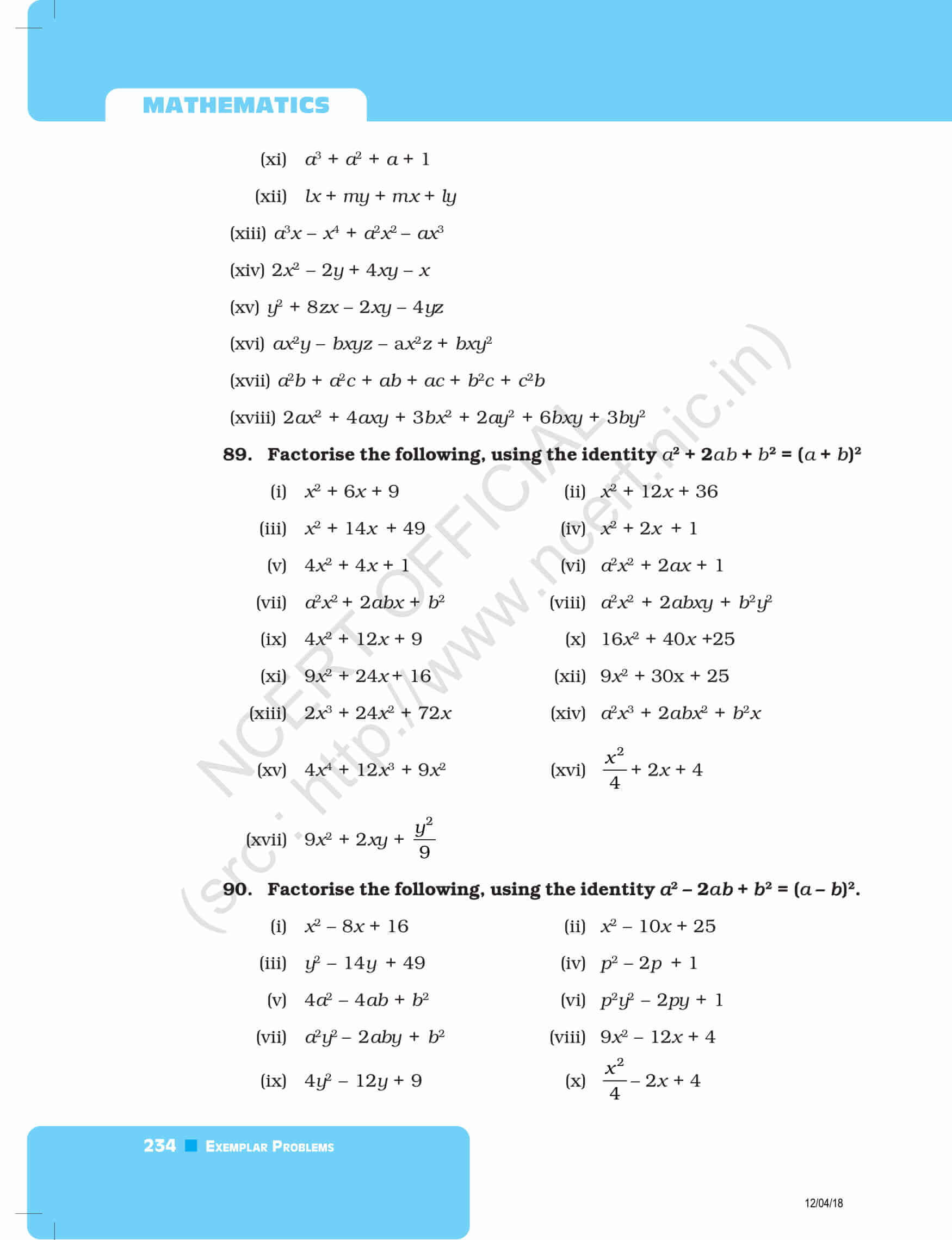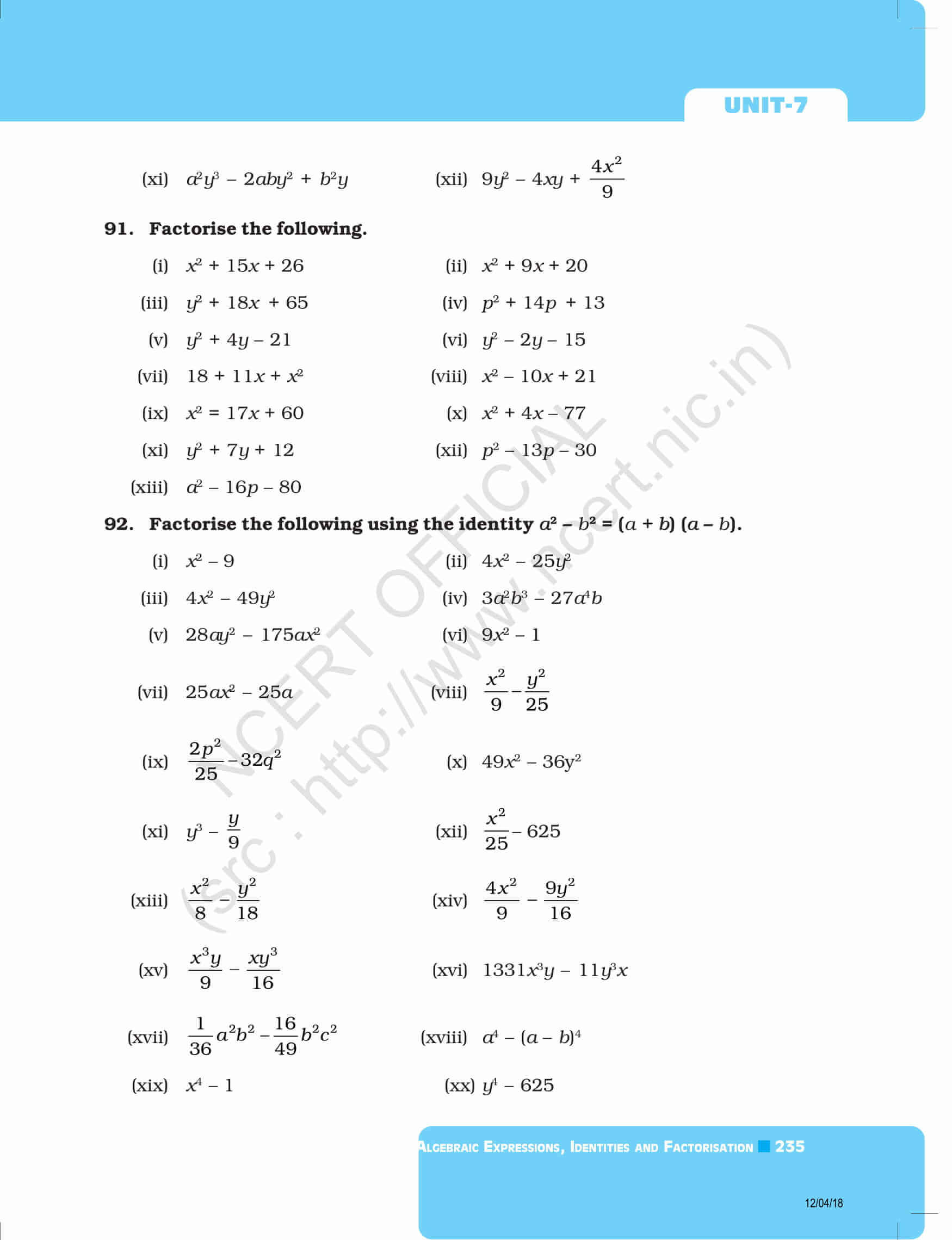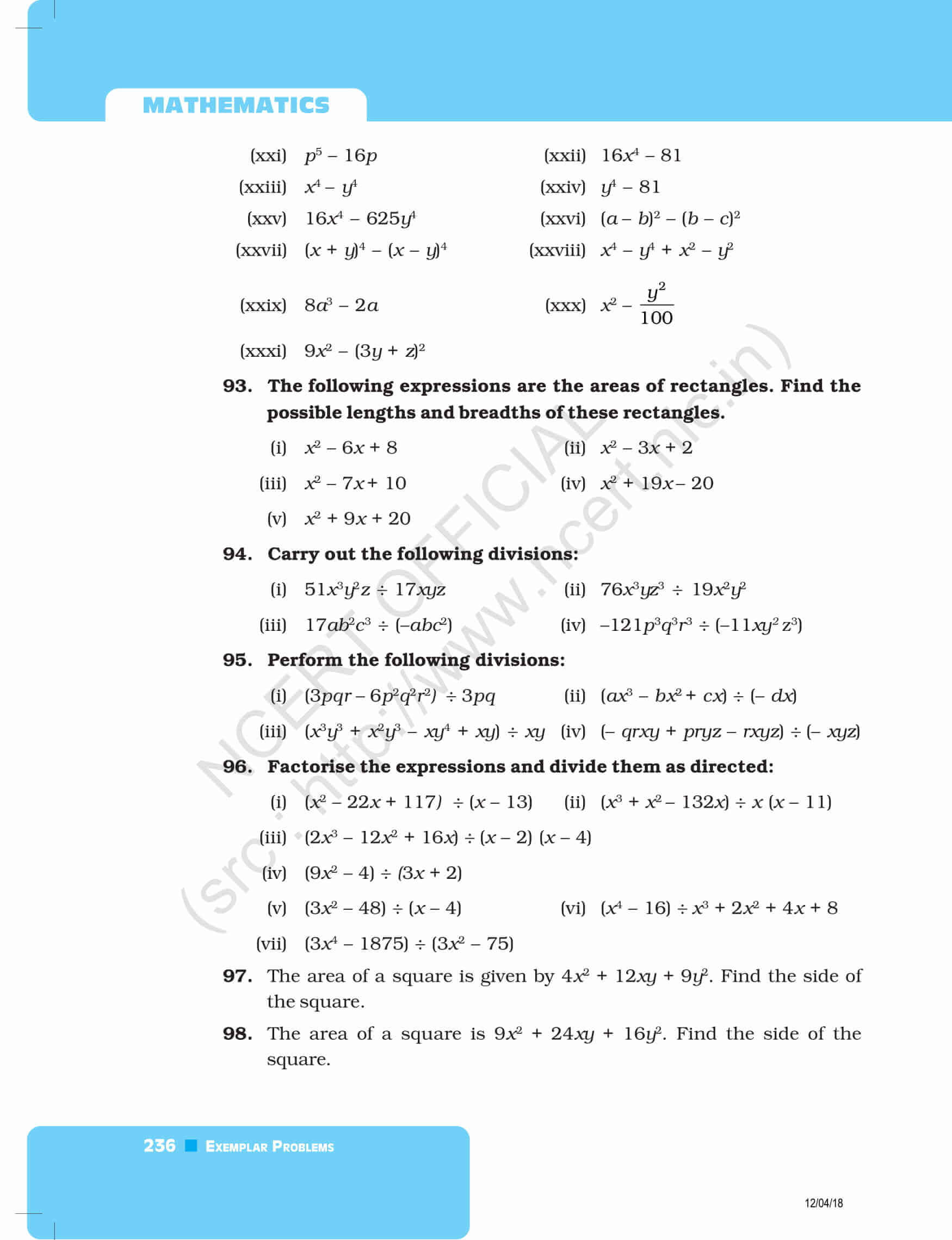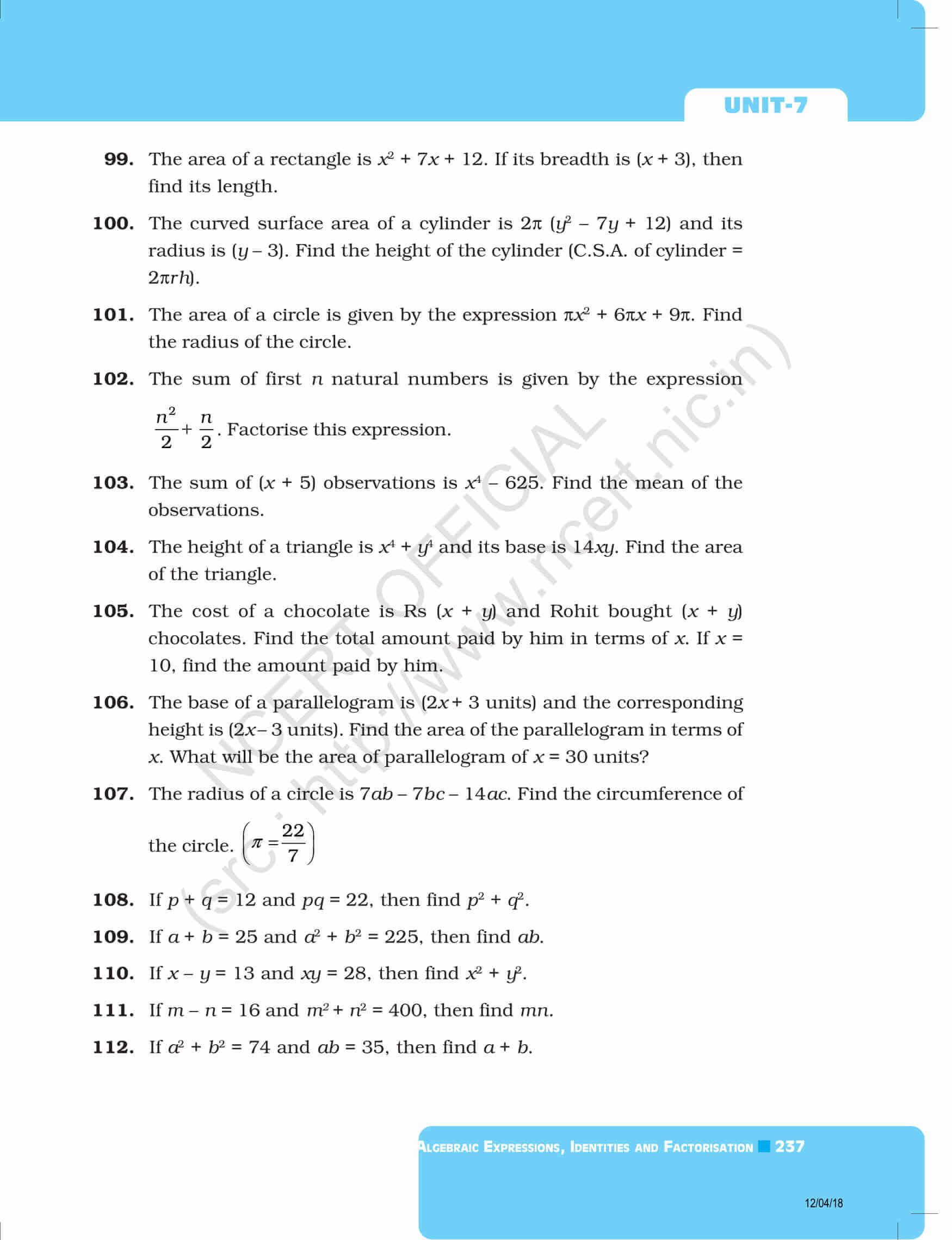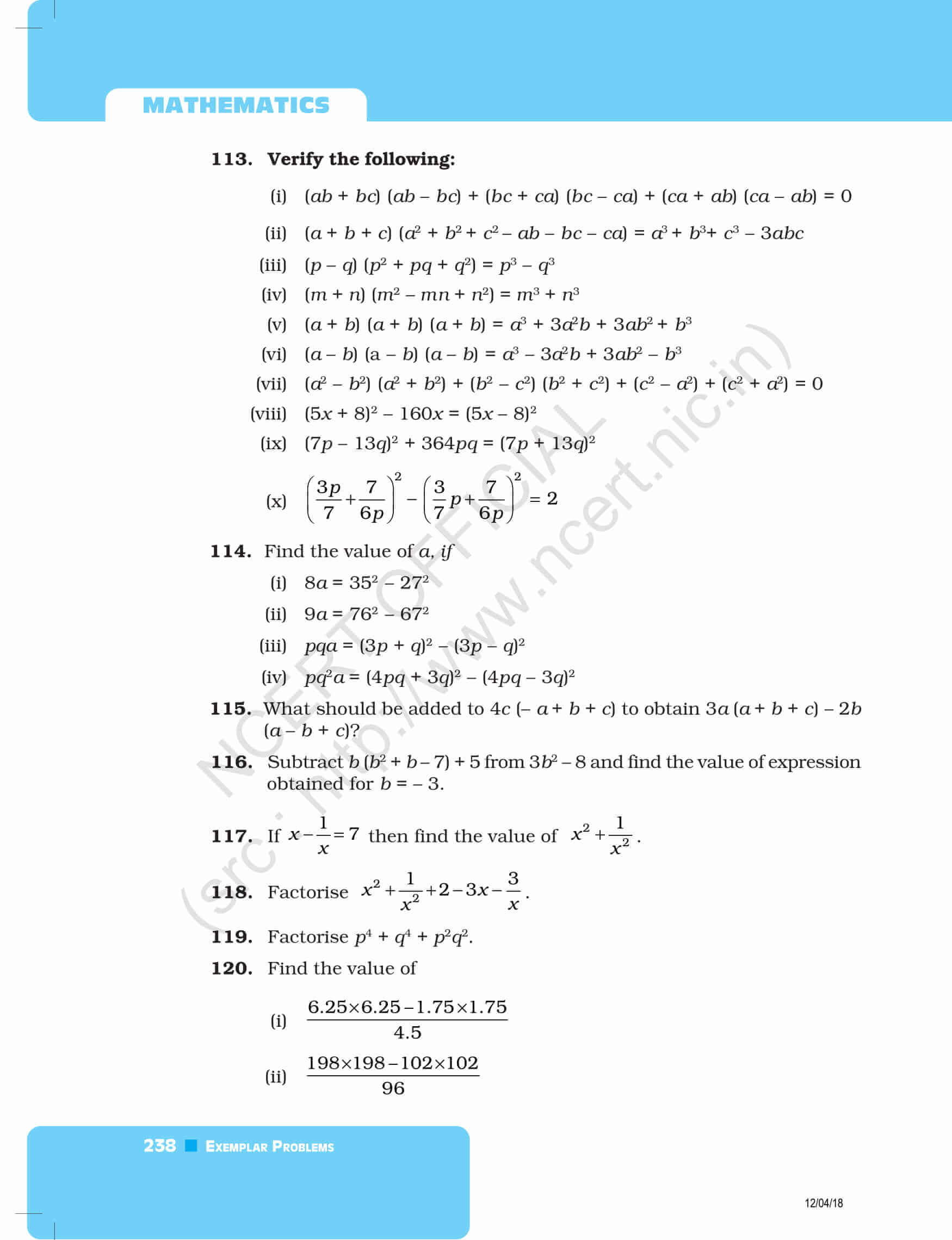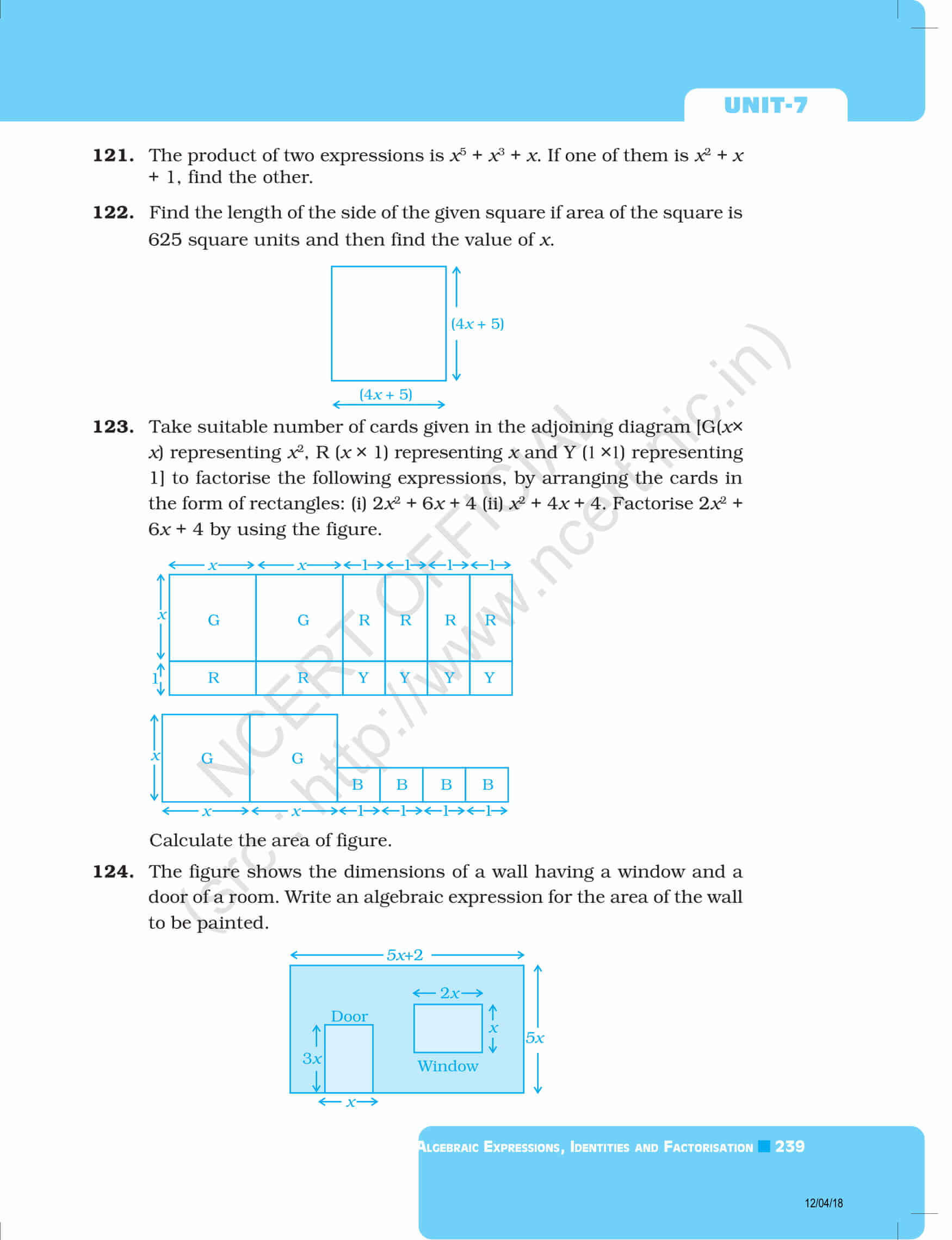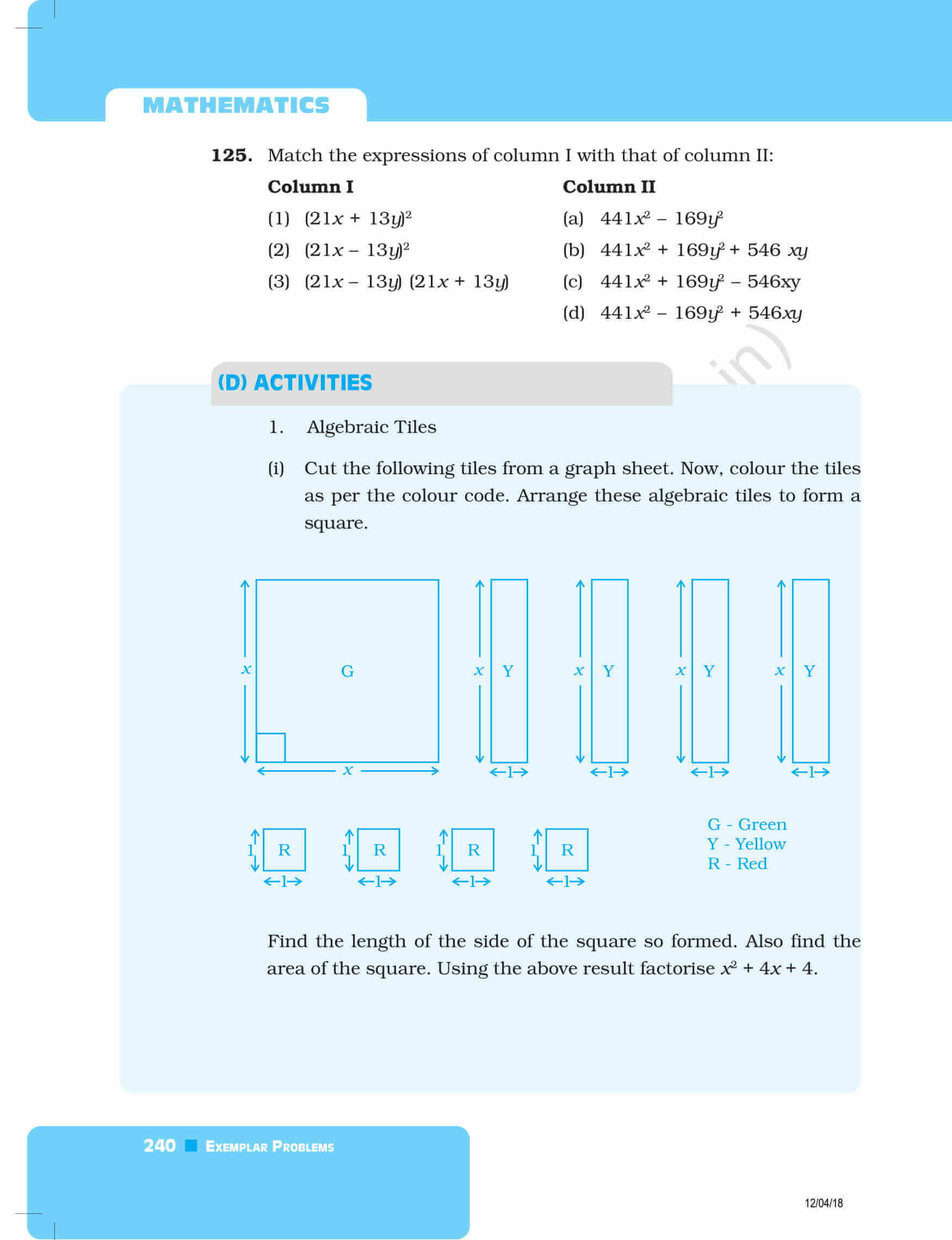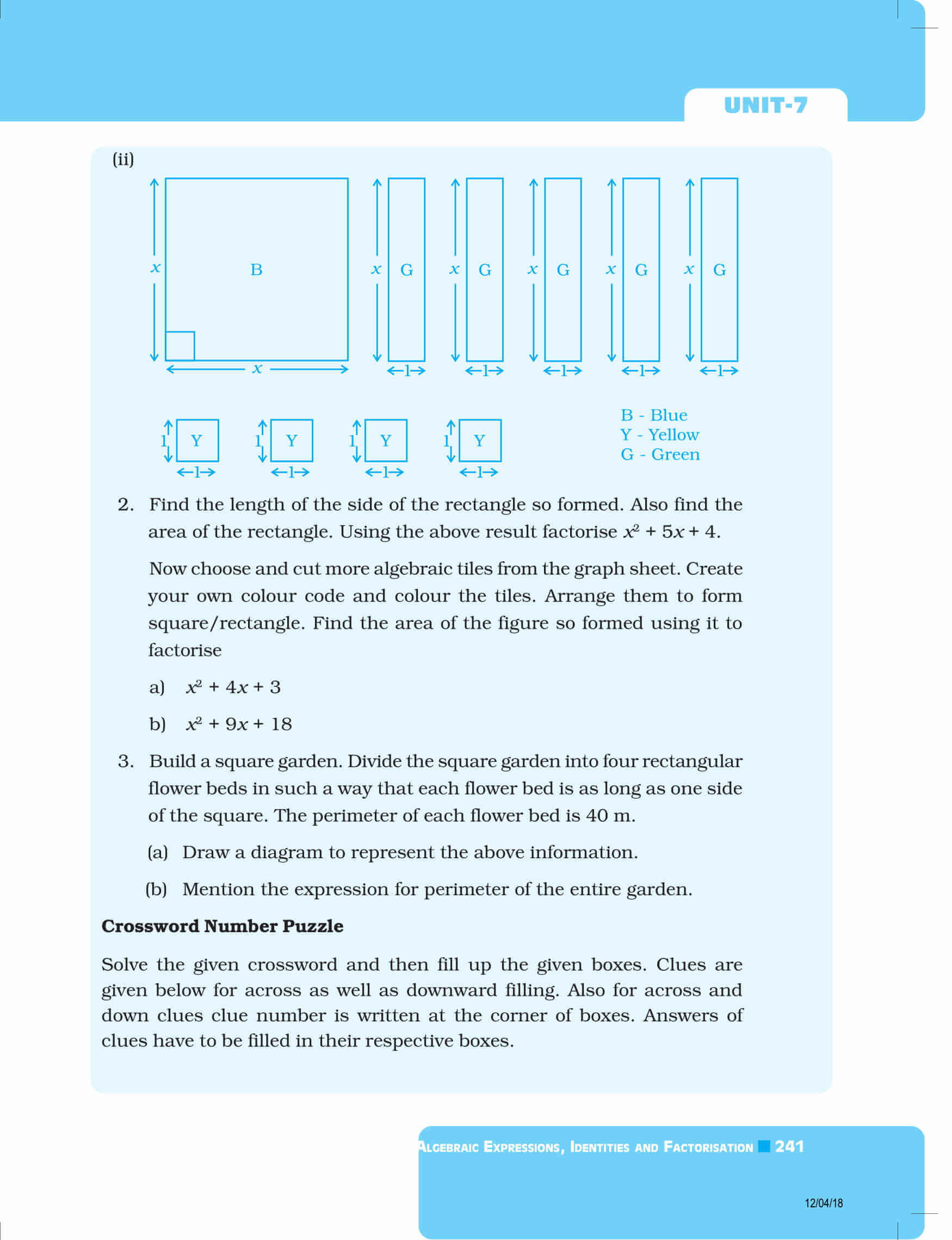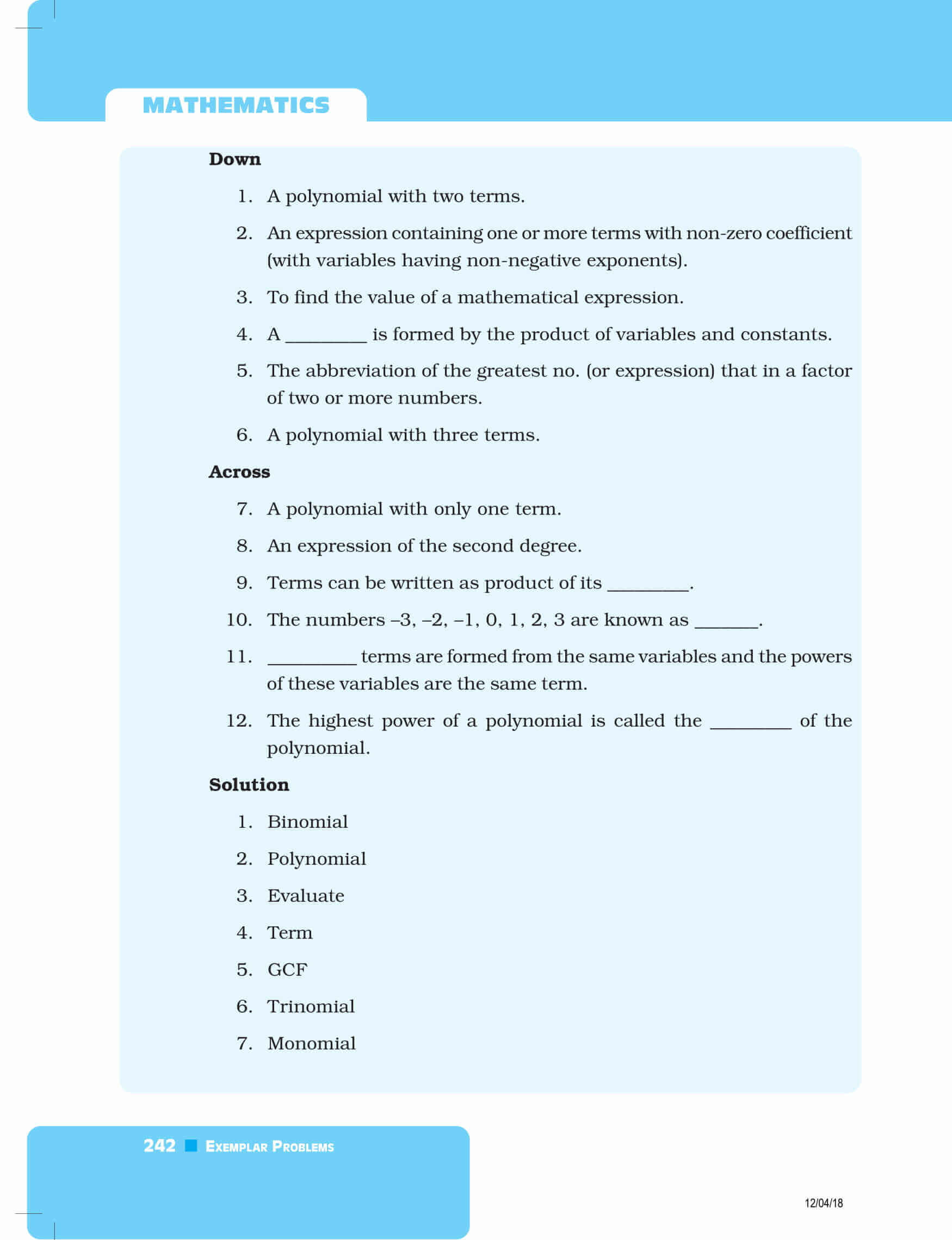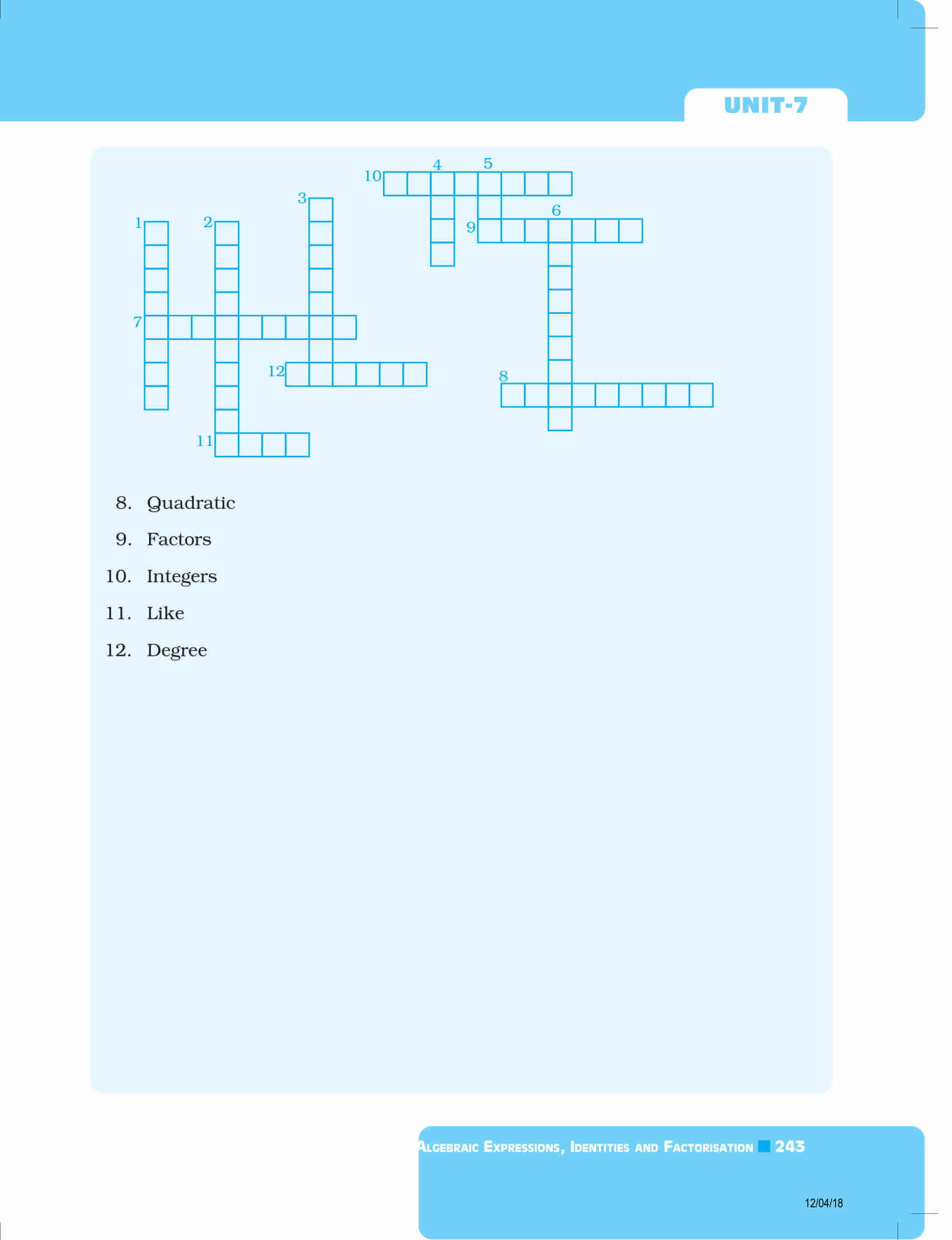Download exemplars solutions for all chapters of 8th class maths by clicking here. Also, download BYJU’S-The Learning App and get personalized videos, explaining the concepts of algebra in terms of expression and identities and their factorisation, with the help of pictures and experience a new way of learning to understand the theories easily.

Also Check